# Lakhmir Singh Solutions Class 10 Physics Chapter 2 Magnetic Effects Of Electric Current

We know that magnetism and electricity are two separated yet interconnected concepts when it comes to electromagnetic force. When these two concepts are brought together they form the basis for electromagnetism.

A moving electric charge can generate a magnetic field while with the help of a magnetic field, electric current can be induced into a conductor. Hans Christian Oersted was the first person to observe that a needle of the compass did not point towards the north pole when it was brought near a wire through which electric current was flowing. With the help of experiments, he was able to conclude that the electric current in the wire produced a magnetic field.

An electromagnet is the best example to explain the relationship between magnetism and electricity. Also, the strength of an electromagnet depends on the number of turns in the coil and the electric current that is flowing through it.

## Download PDF Of Lakhmir Singh Physics Class 10 Solutions For Chapter 2 Magnetic Effects Of Electric Current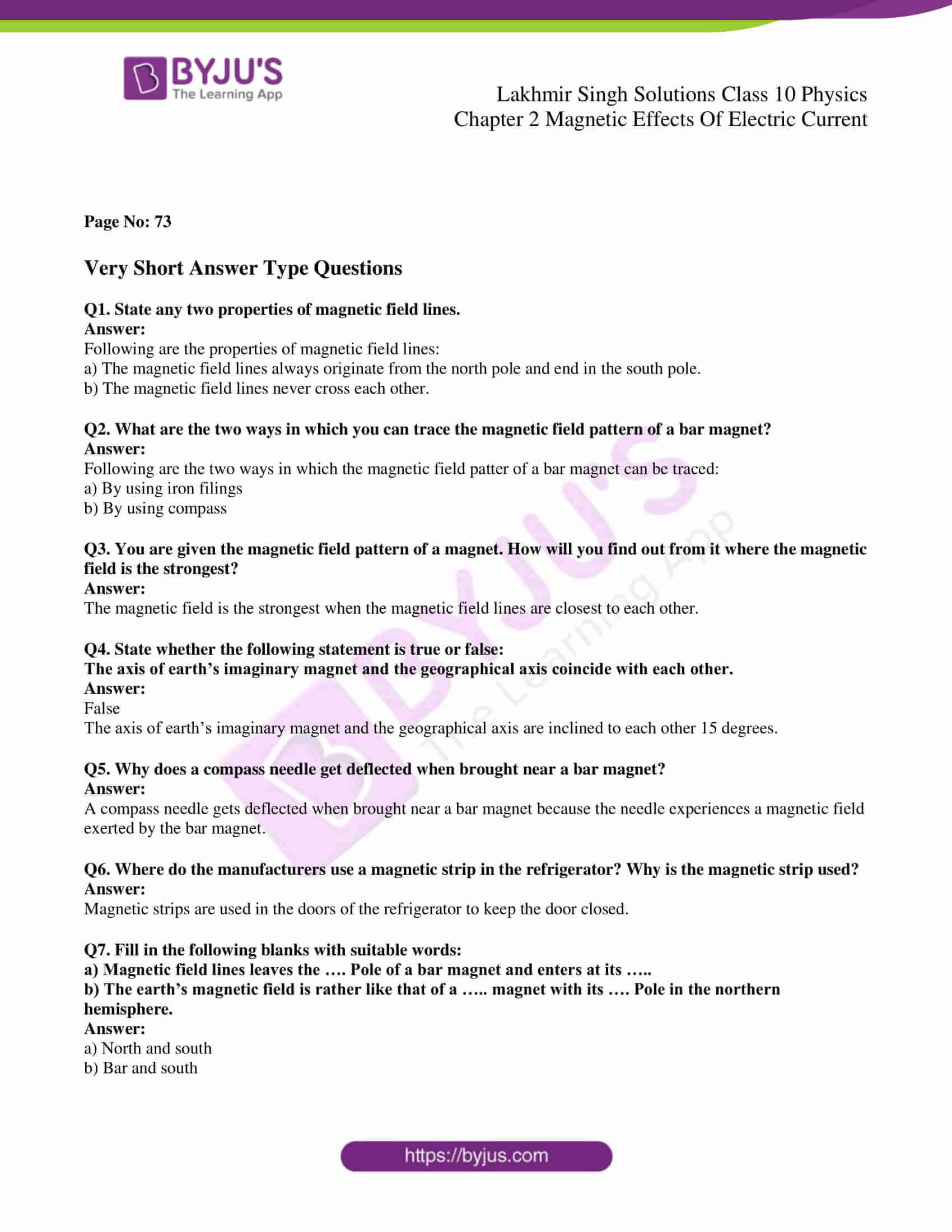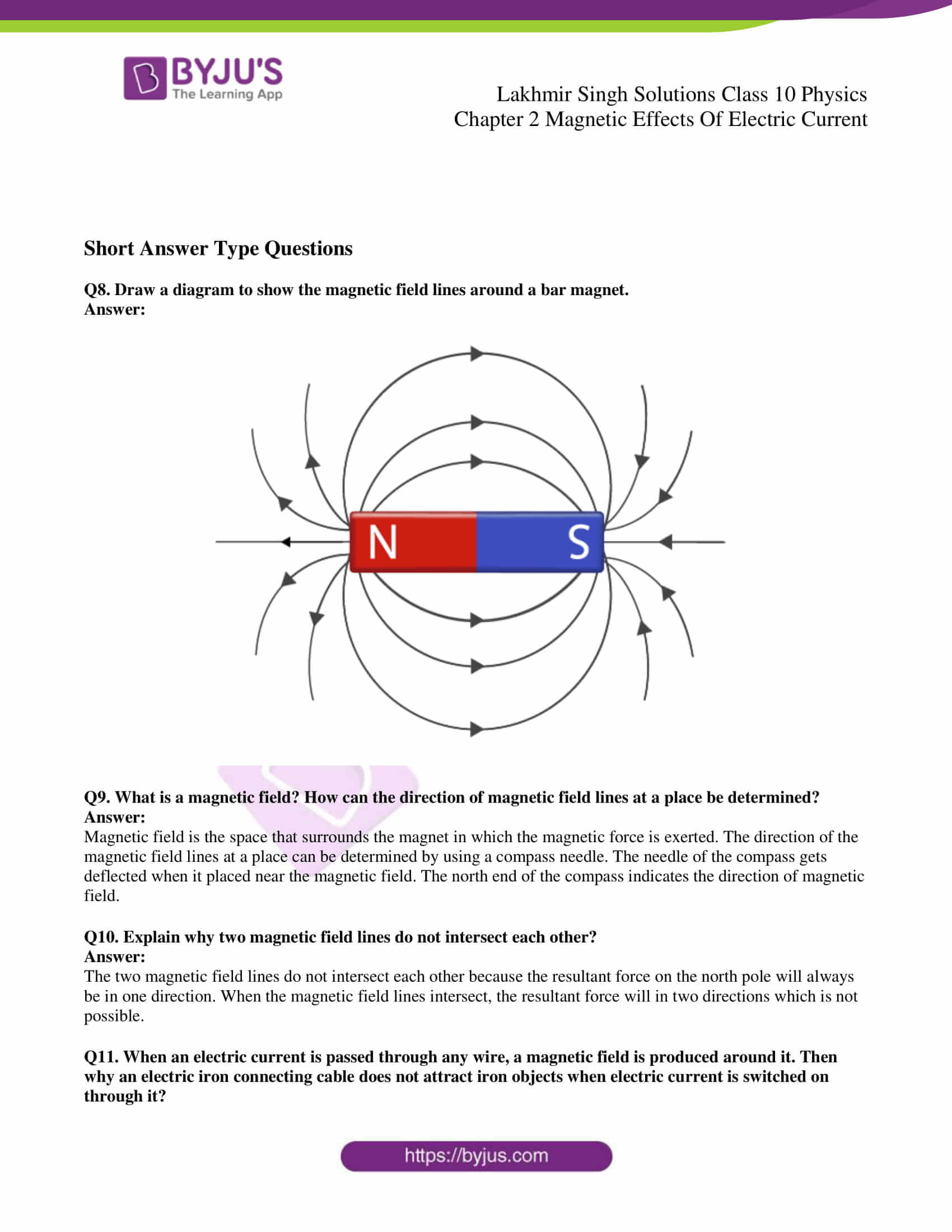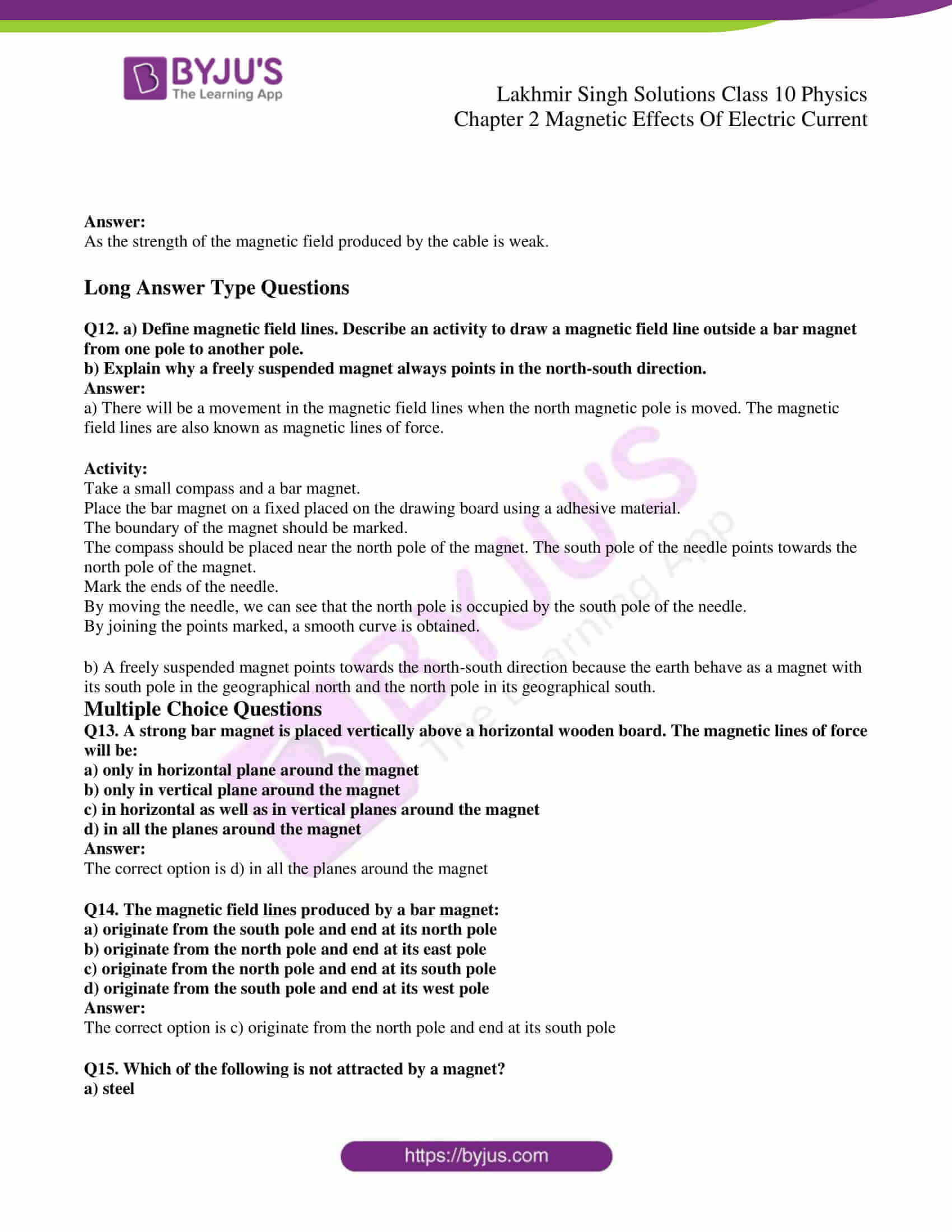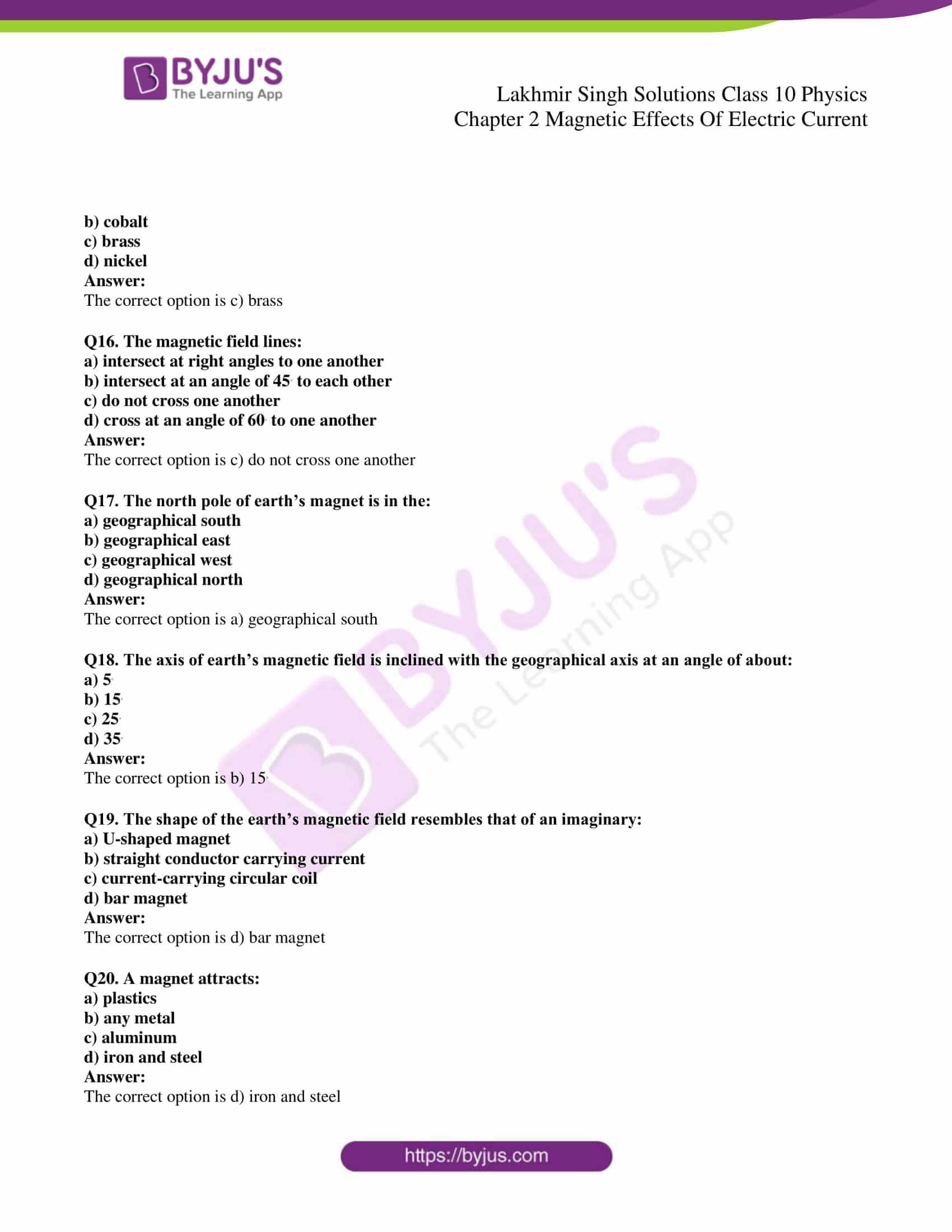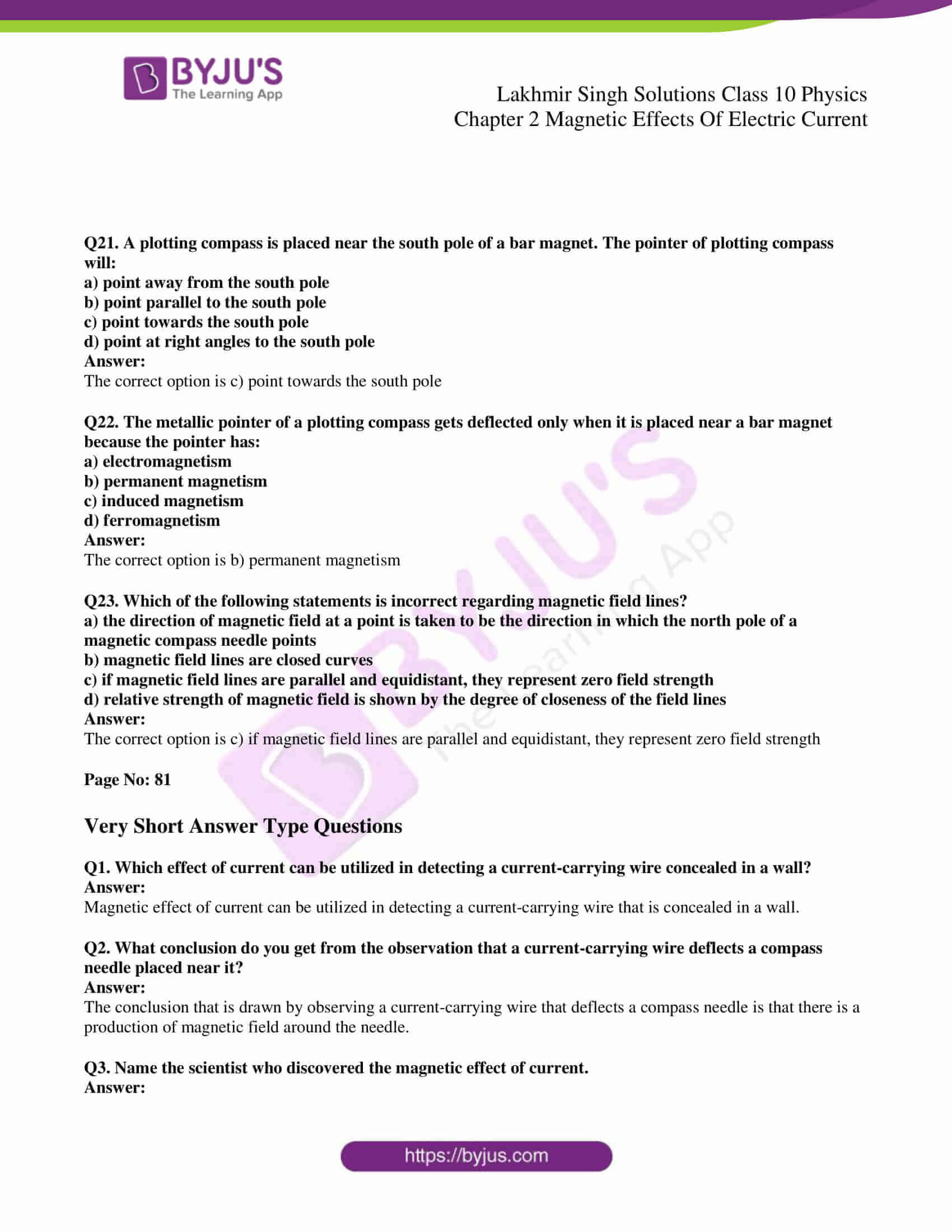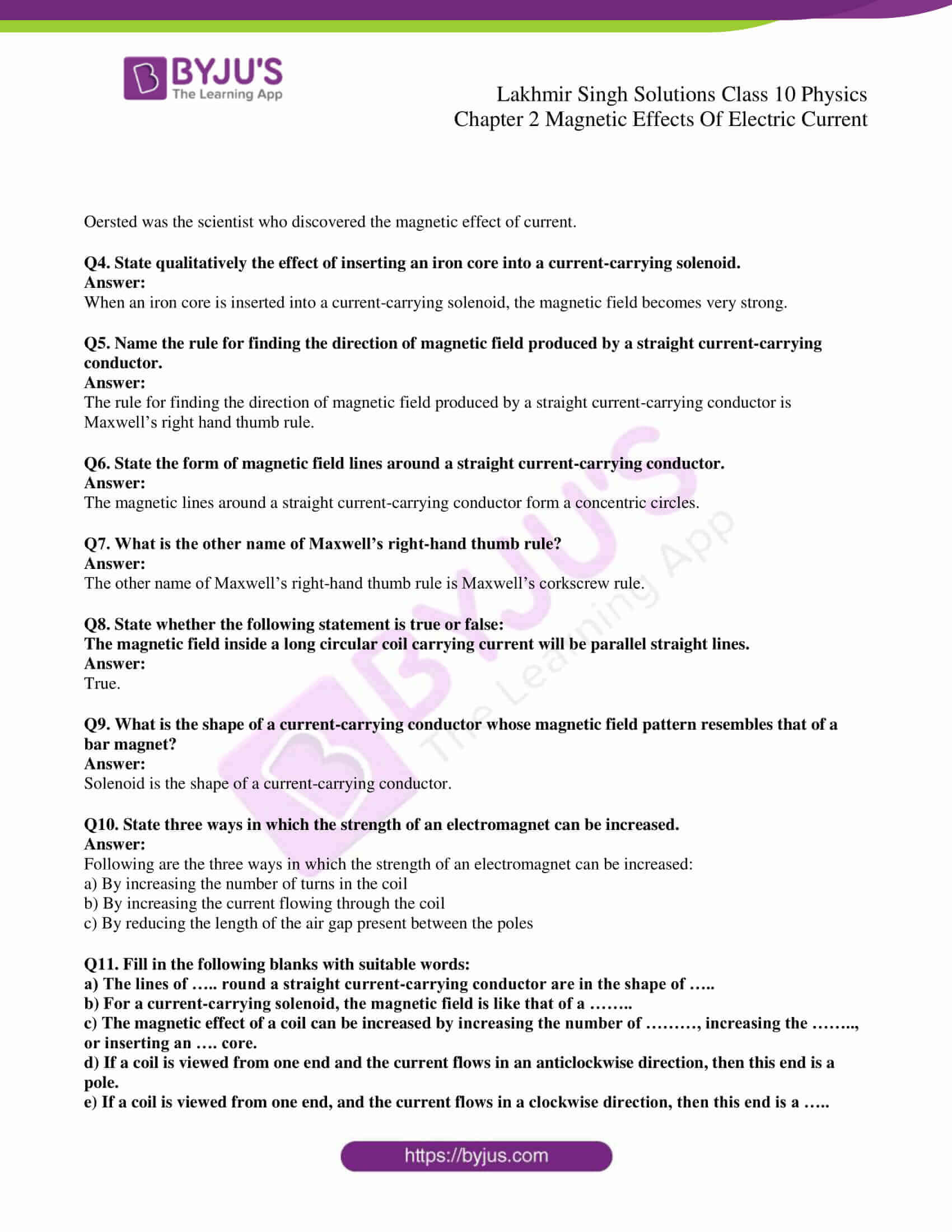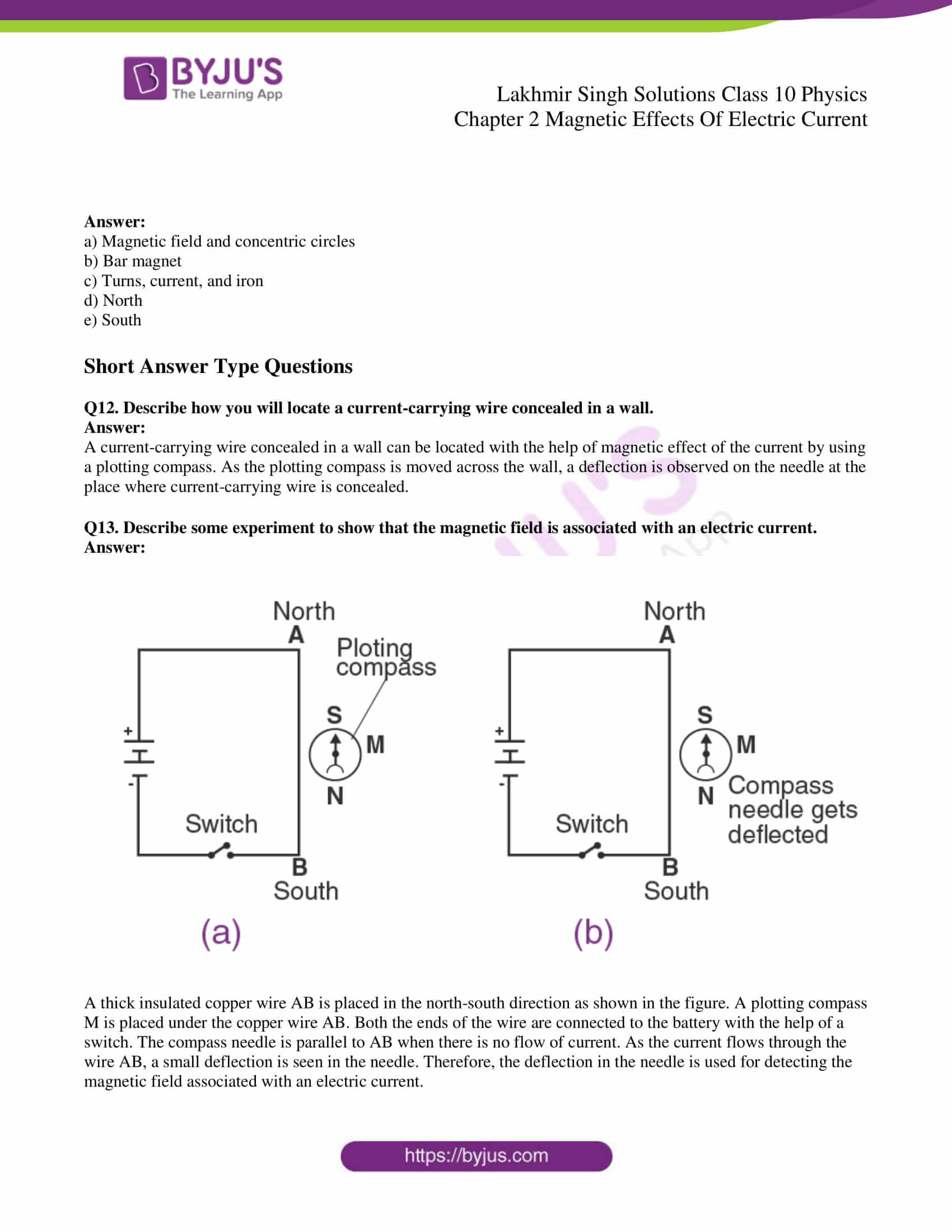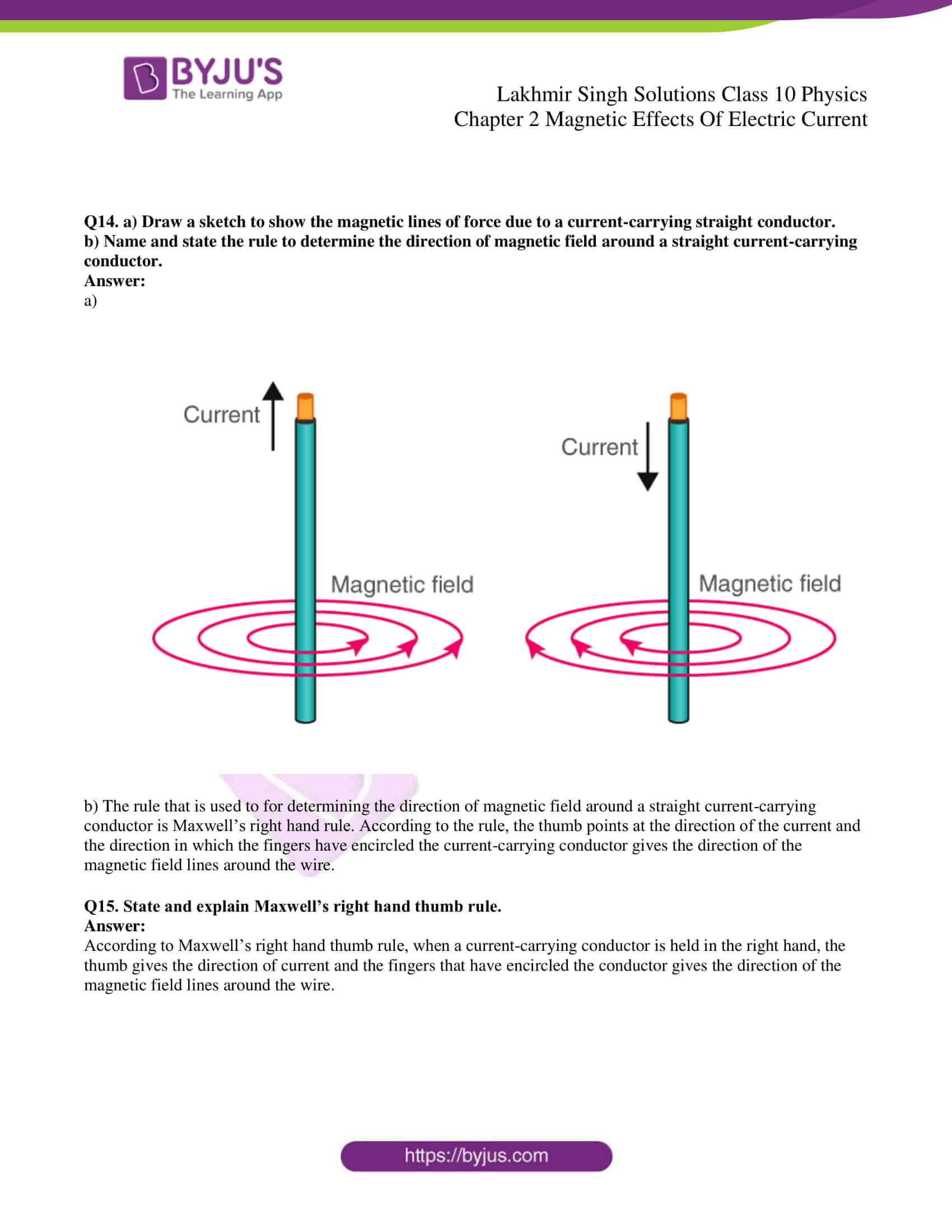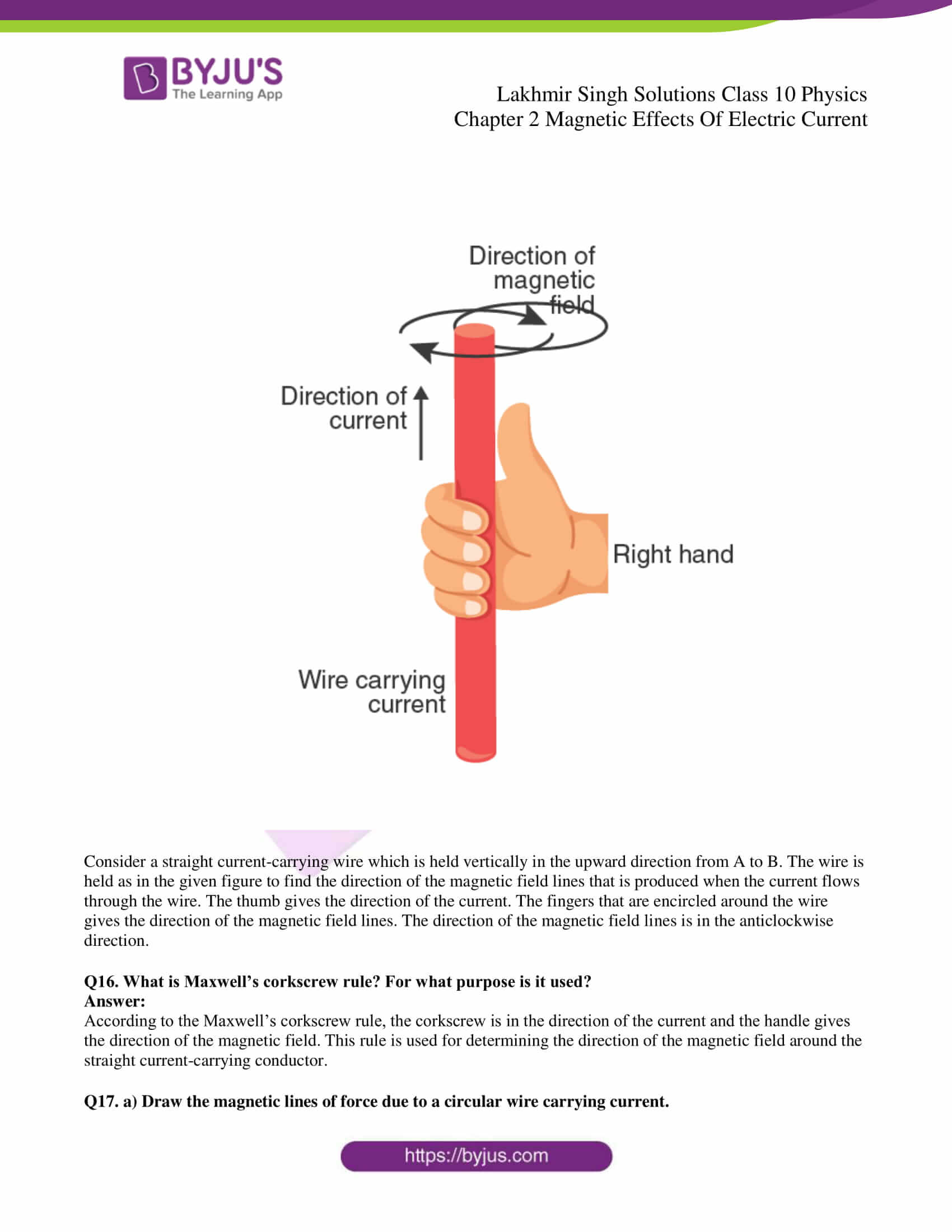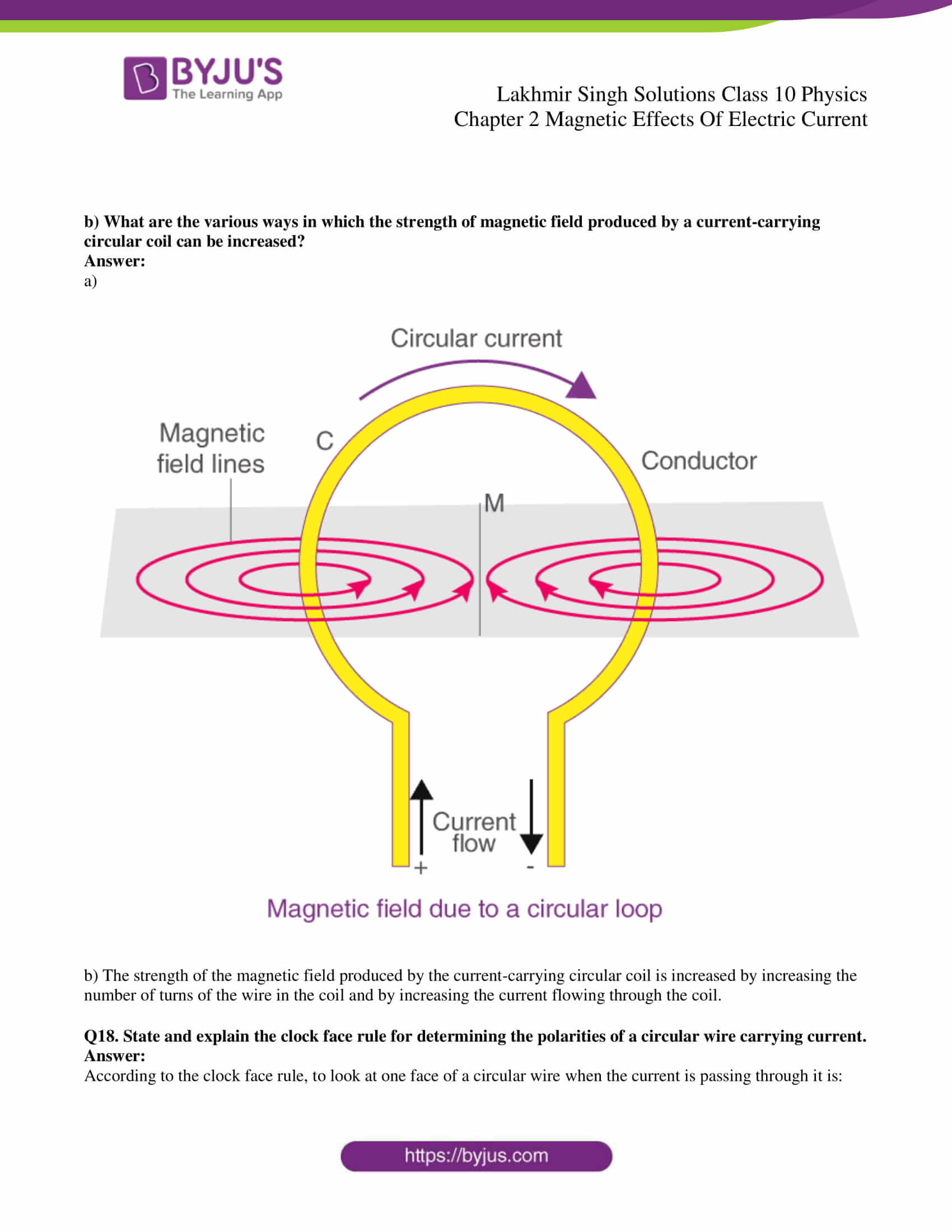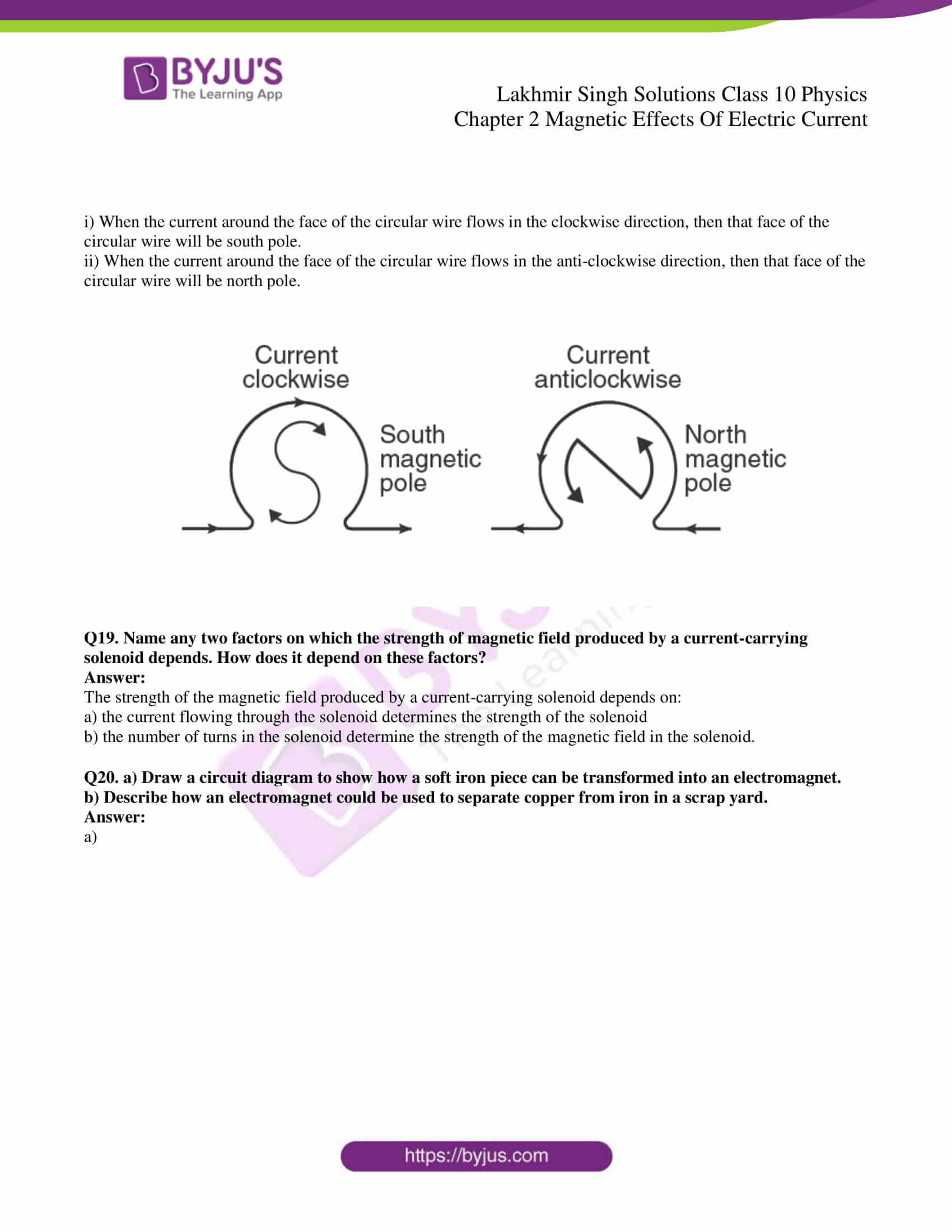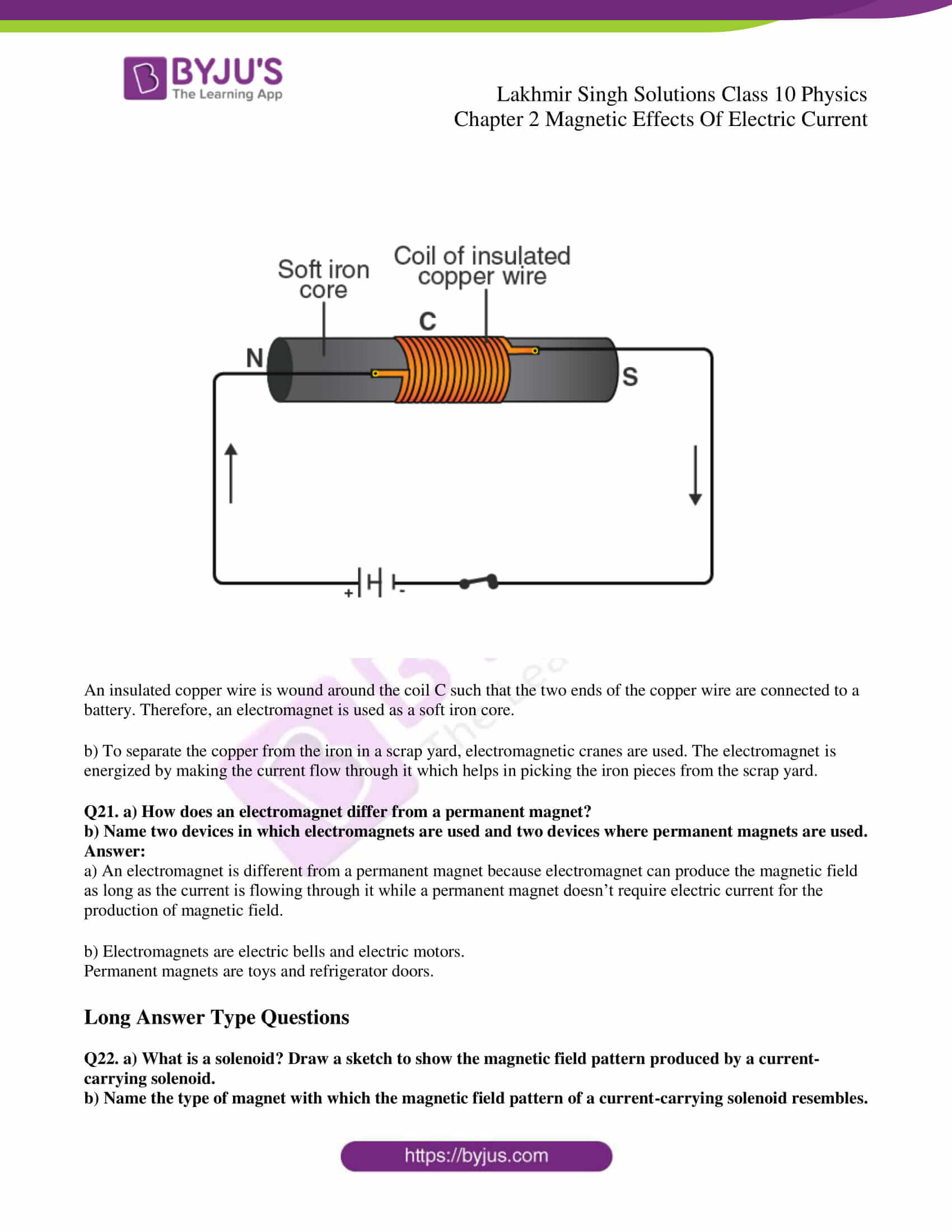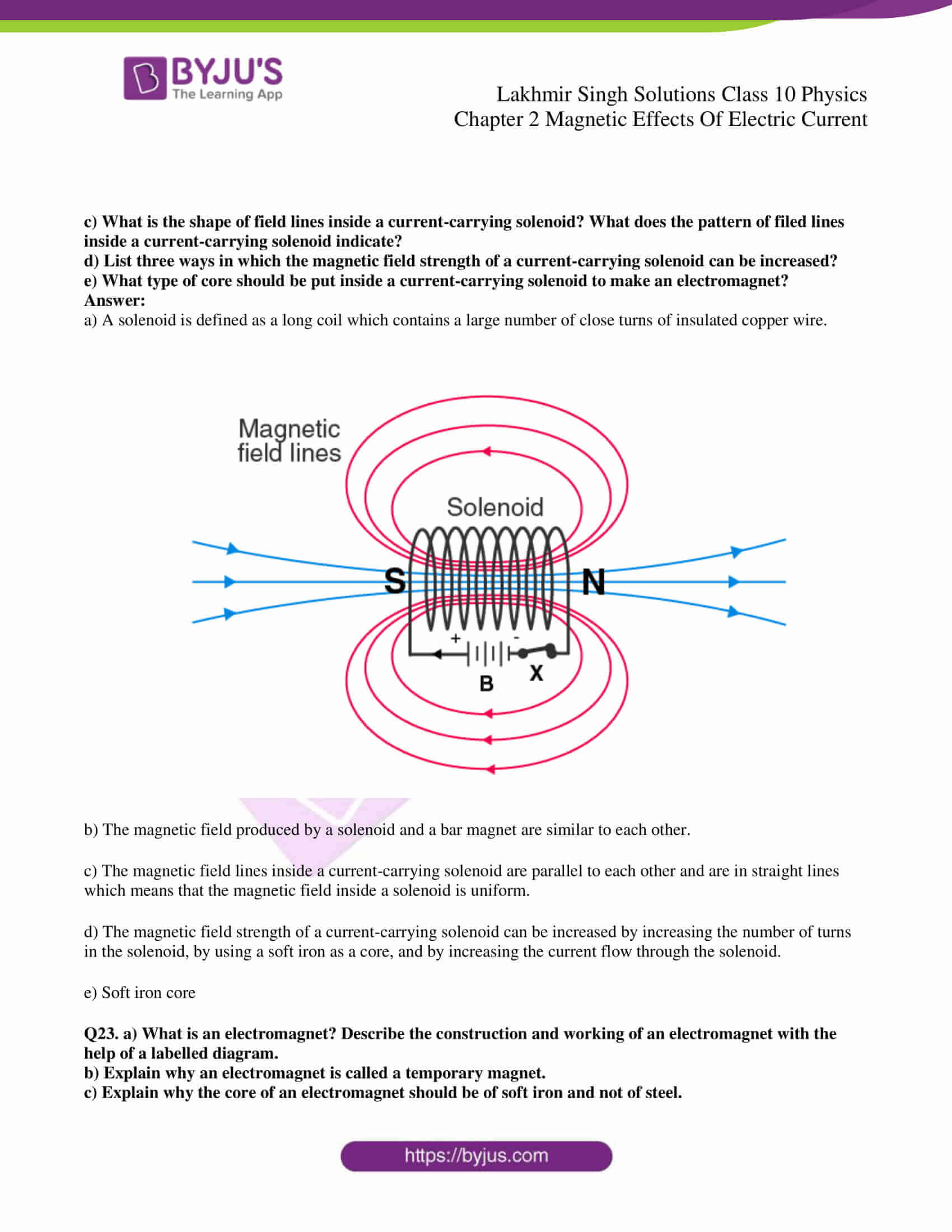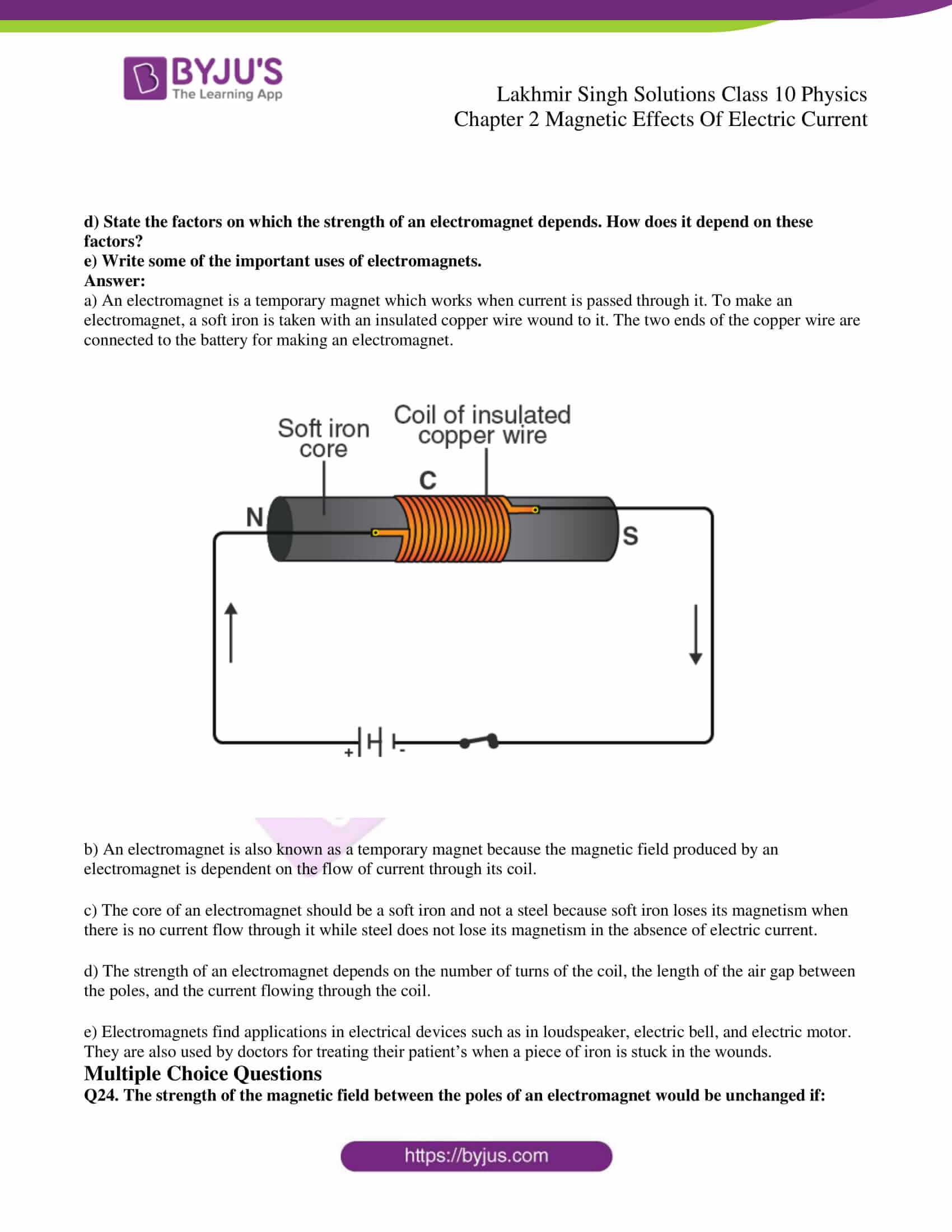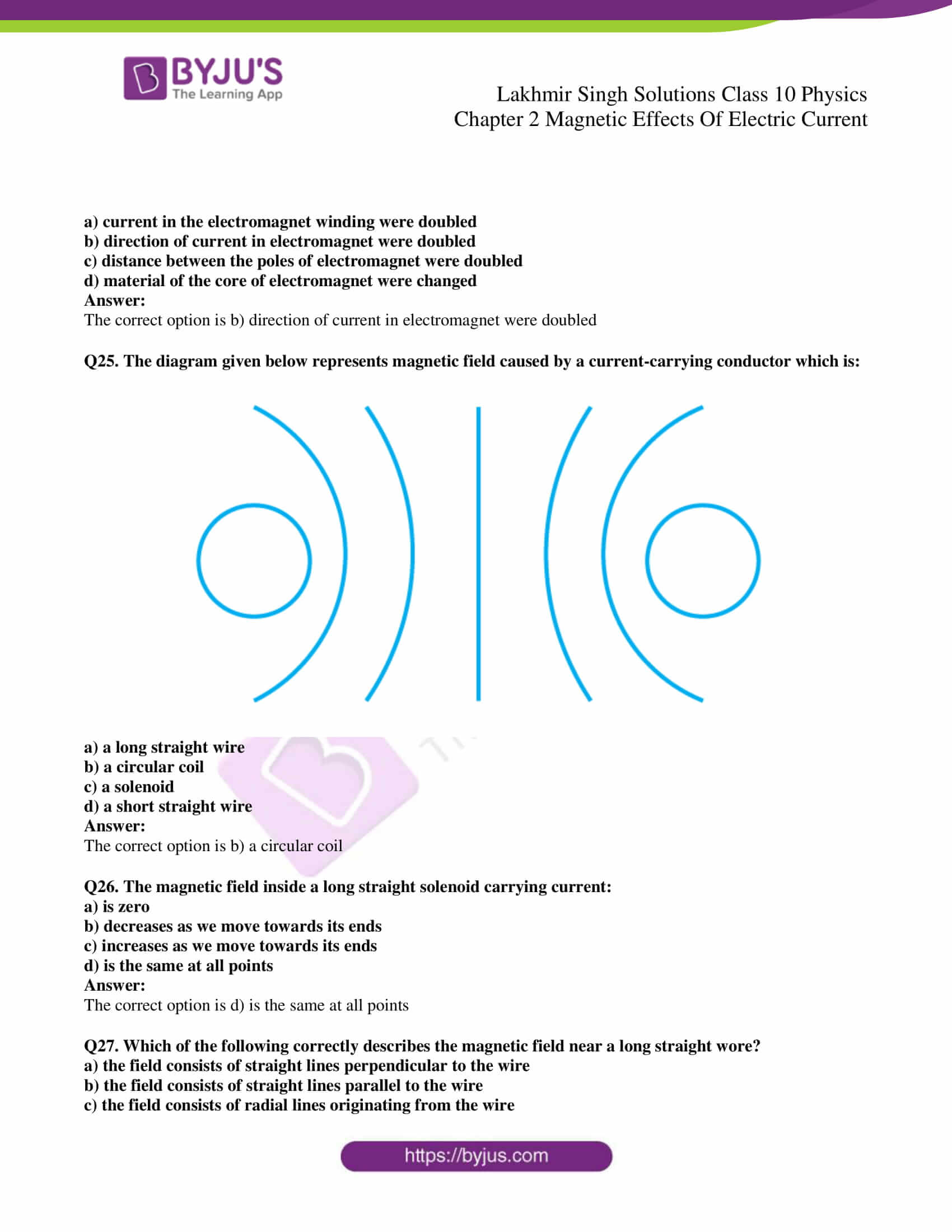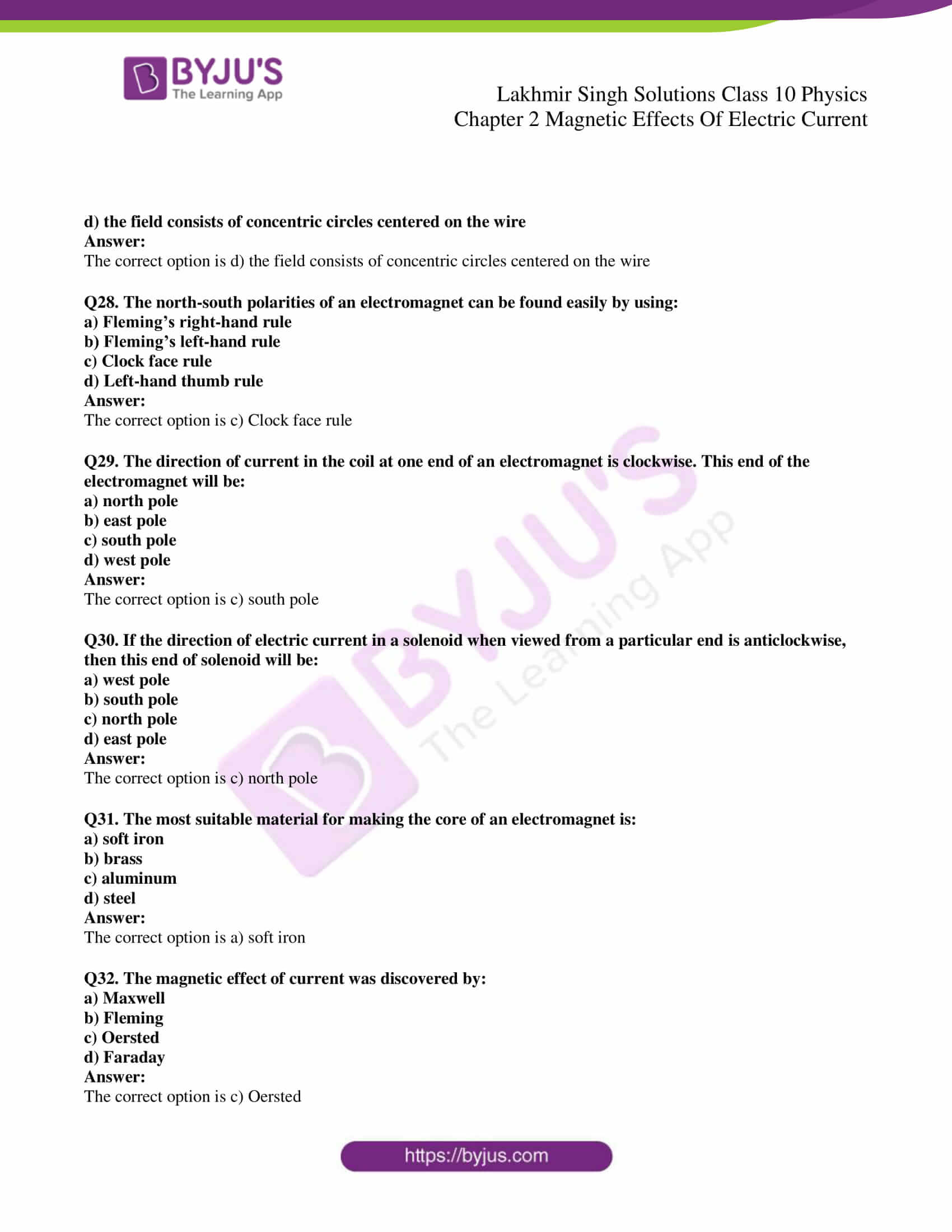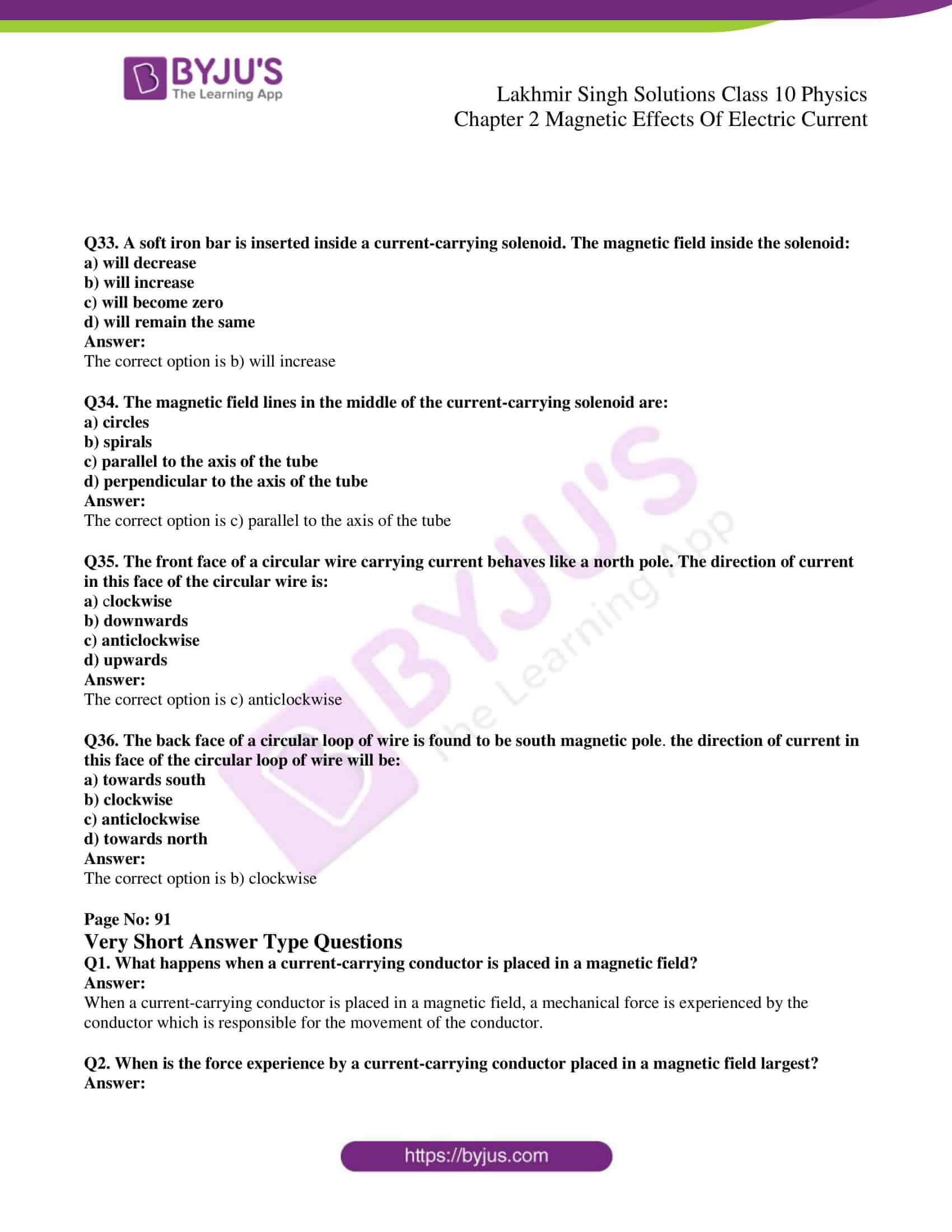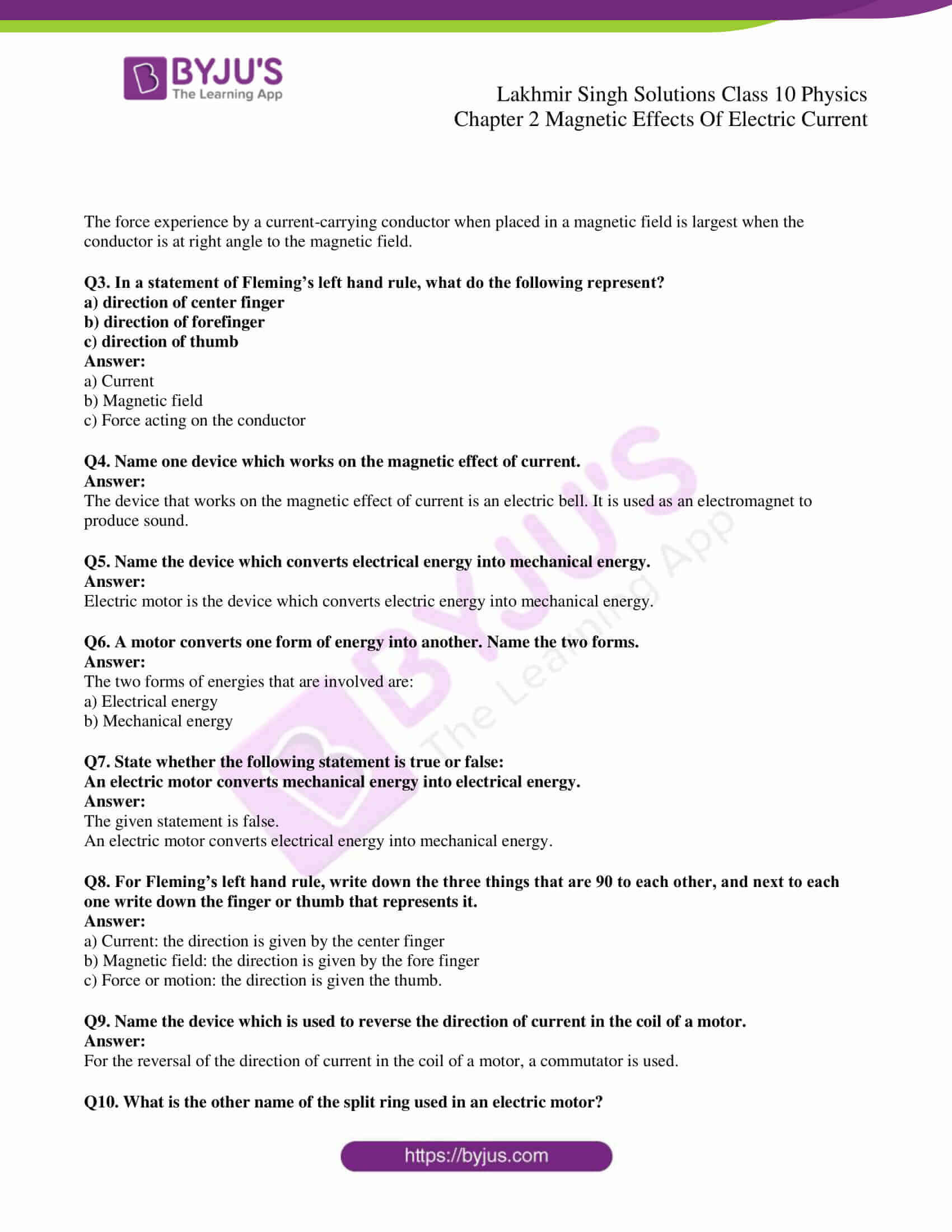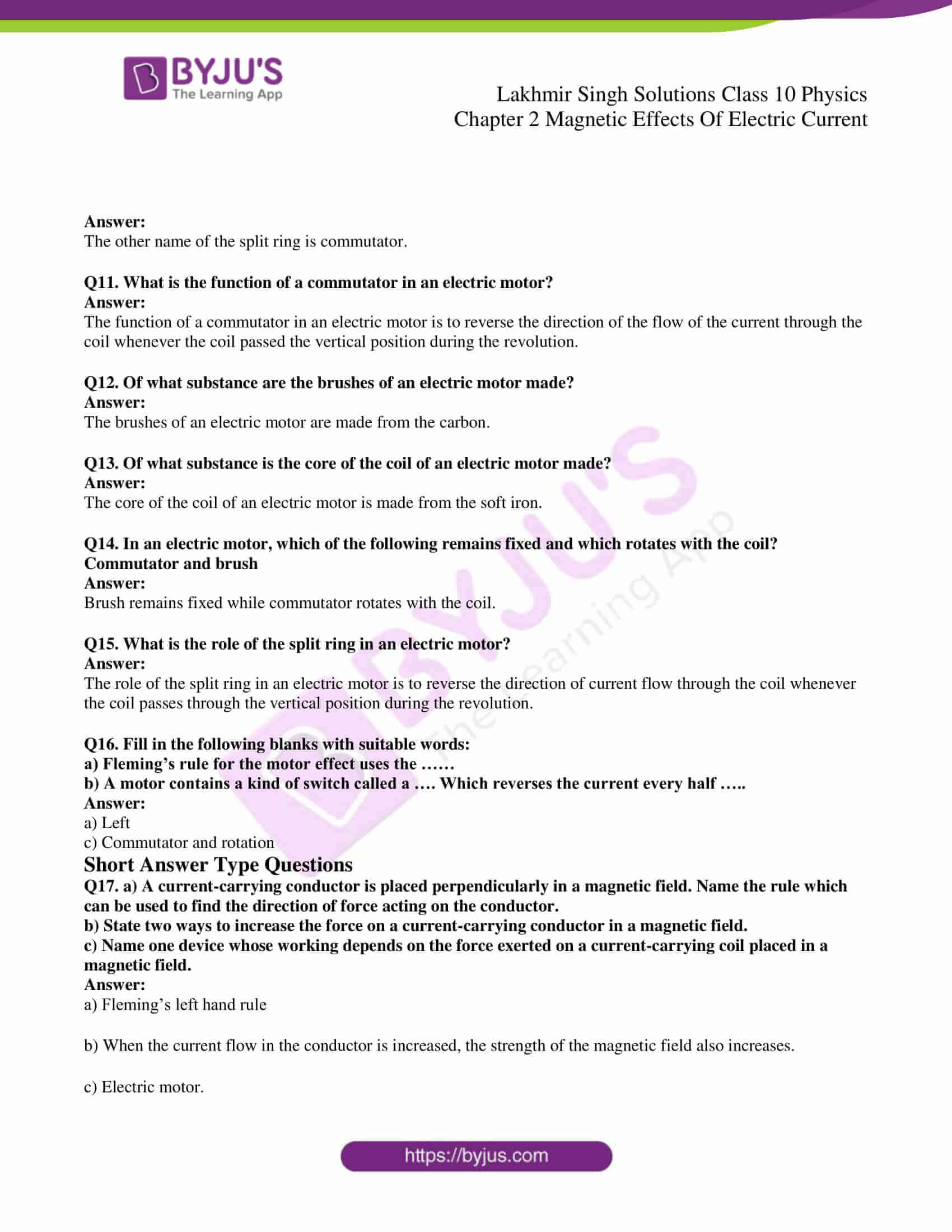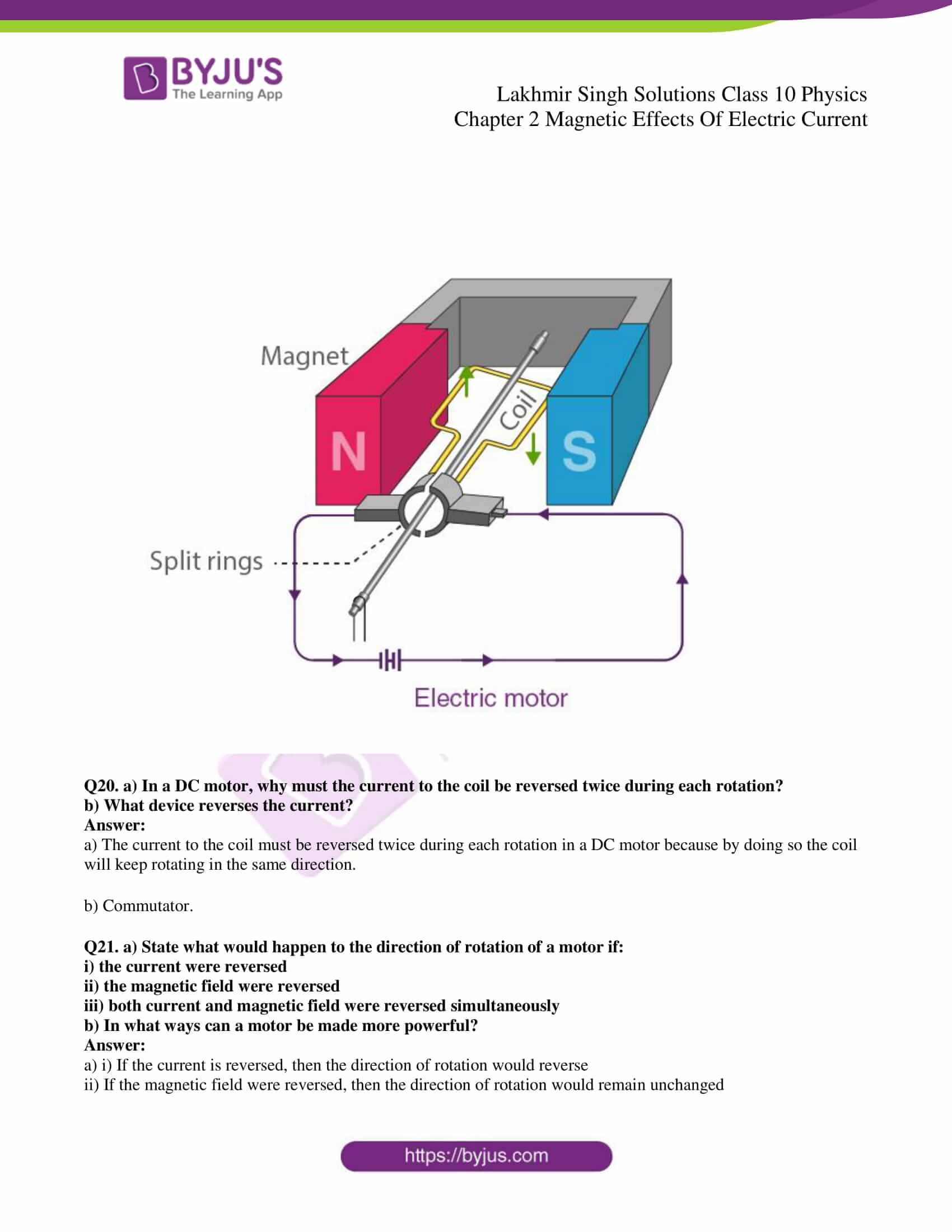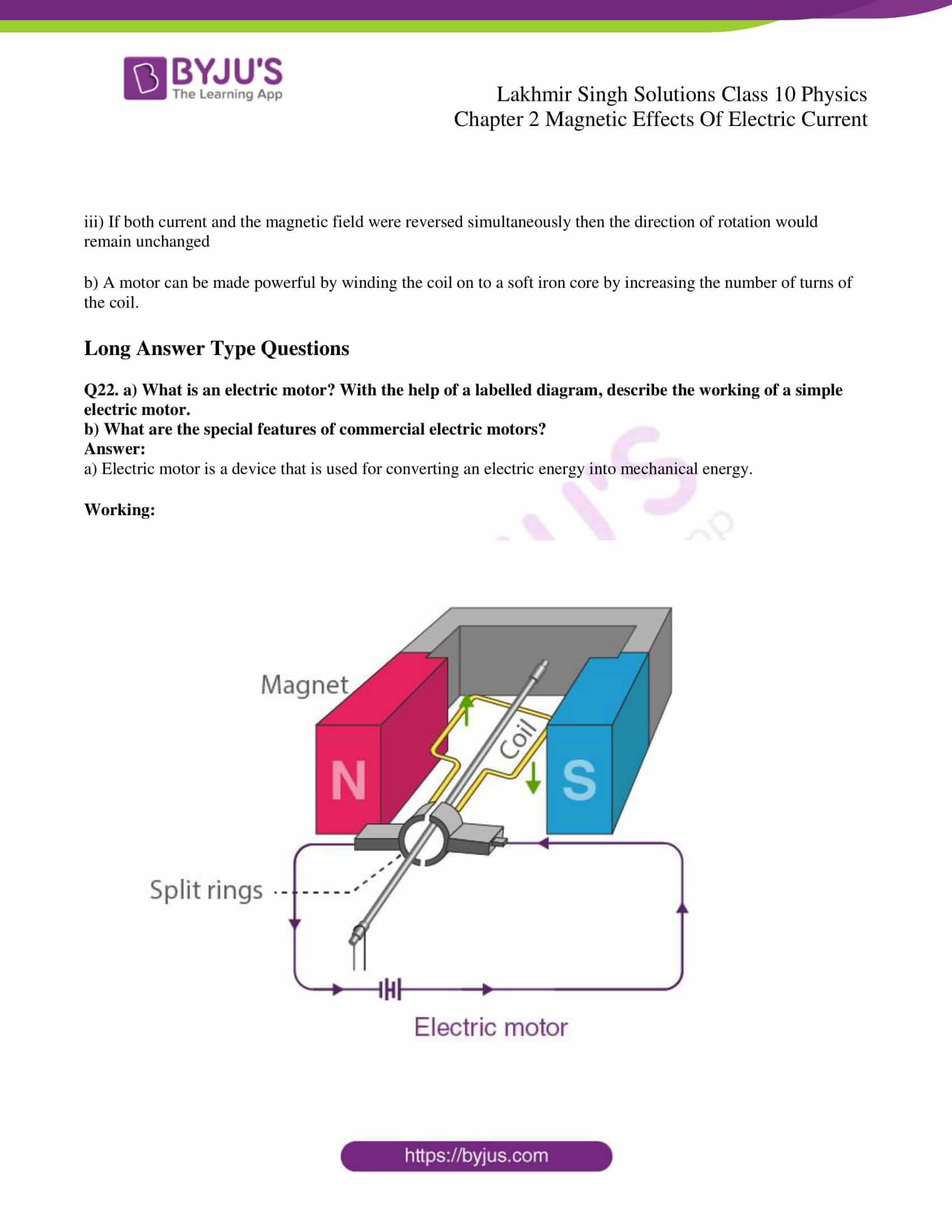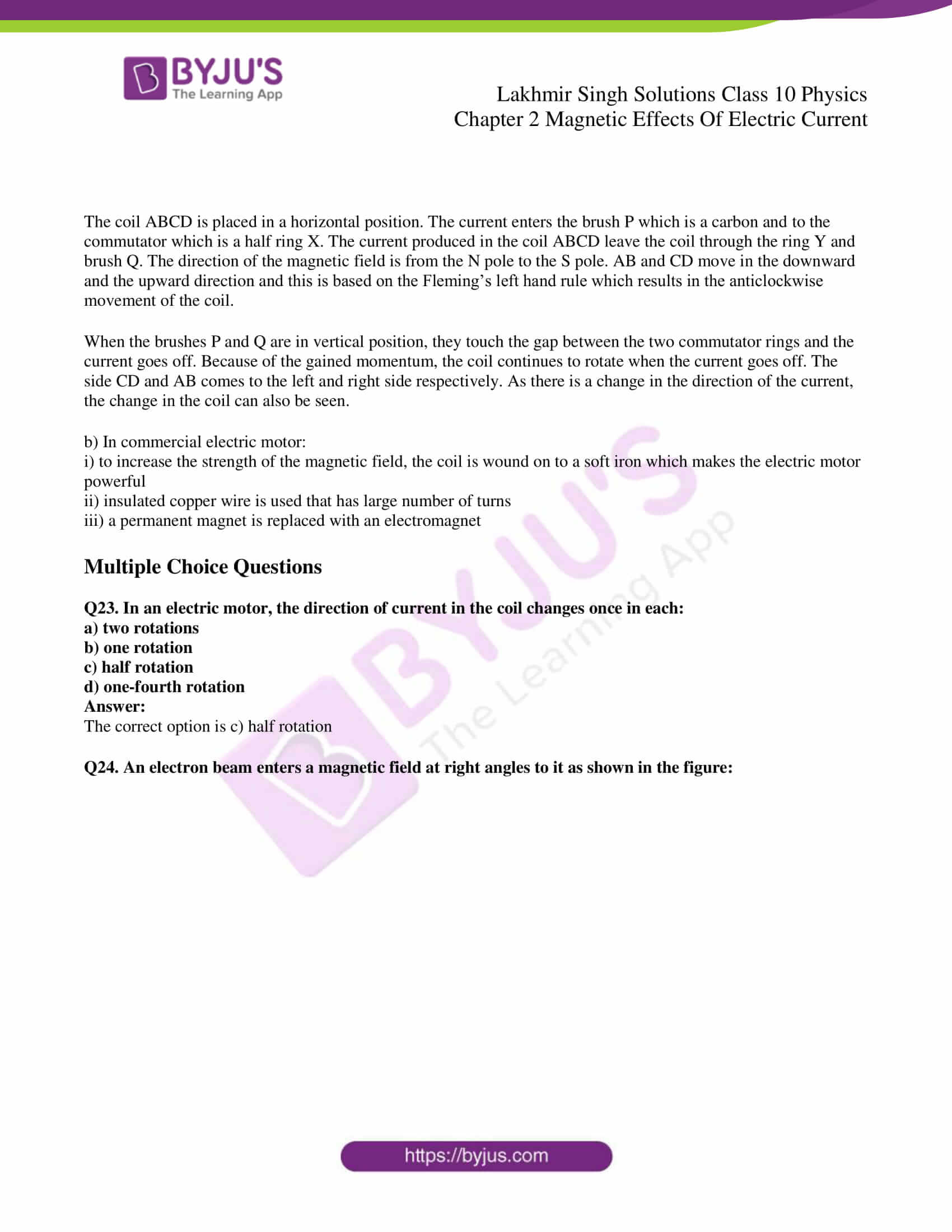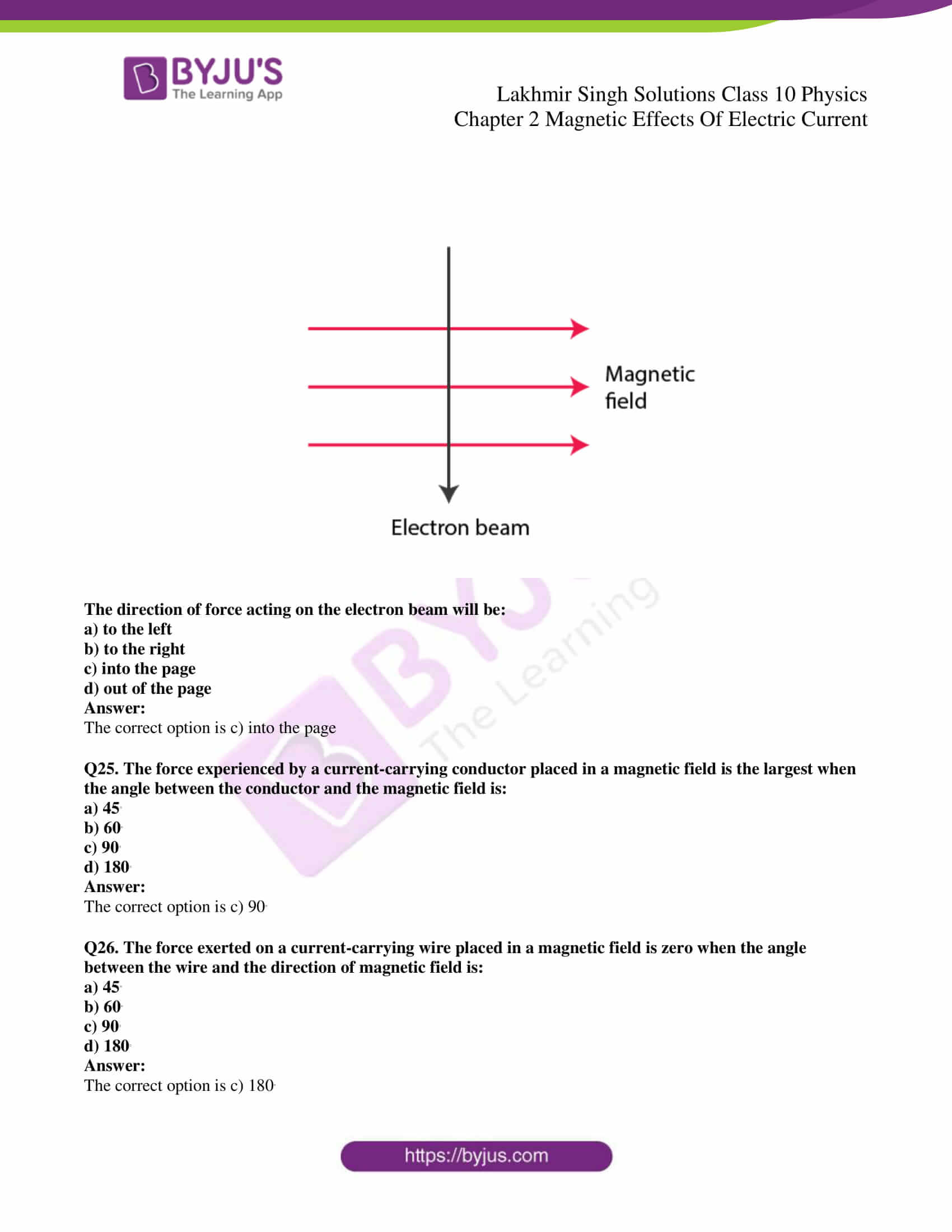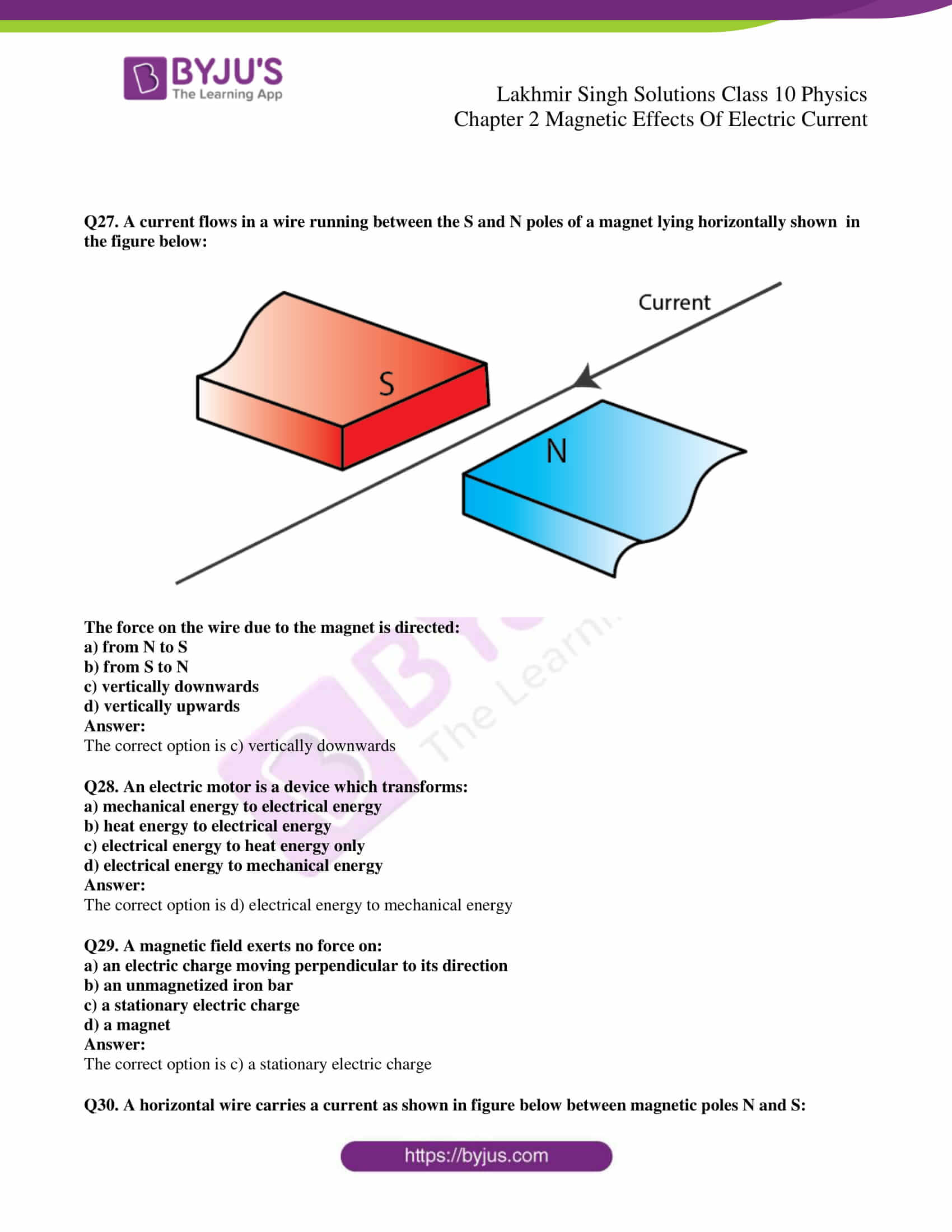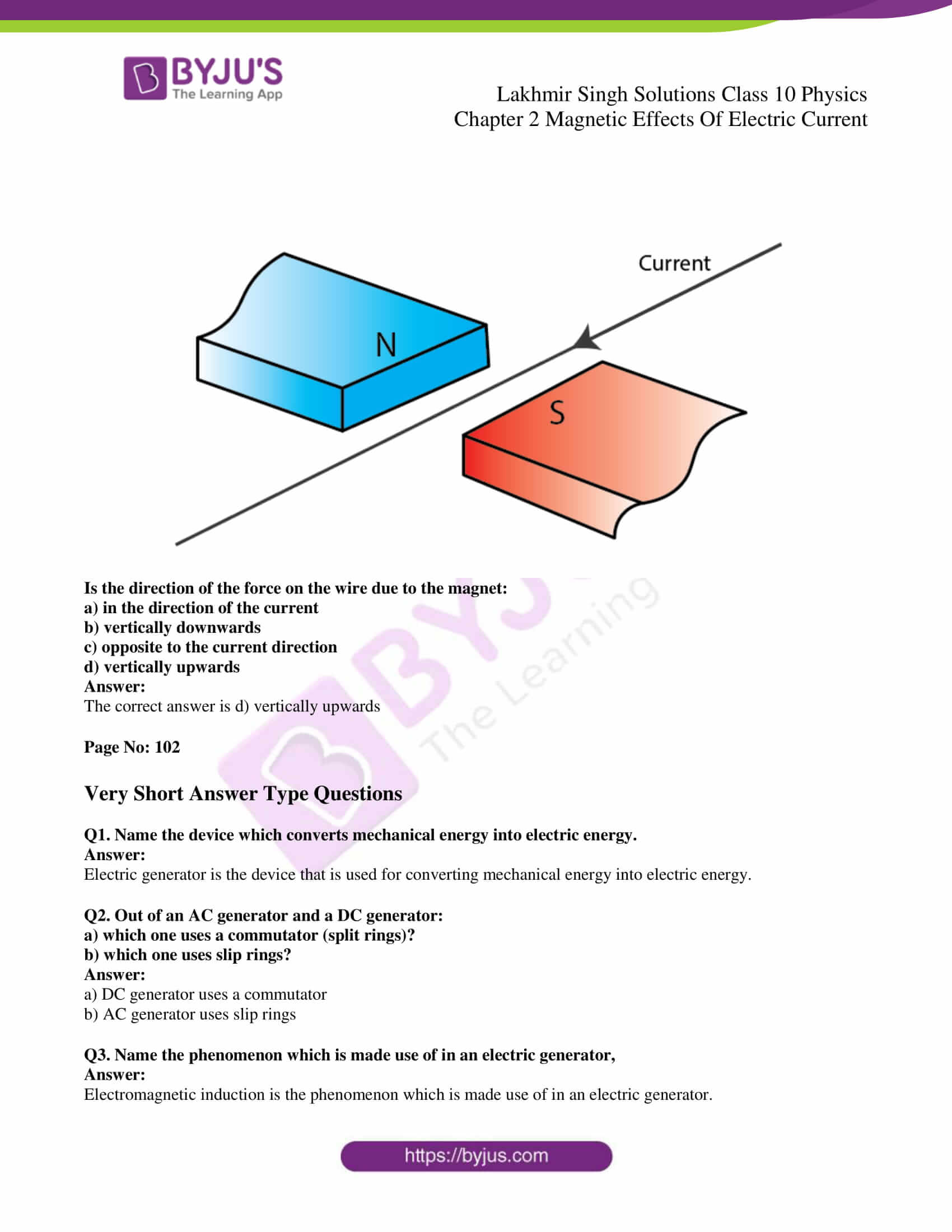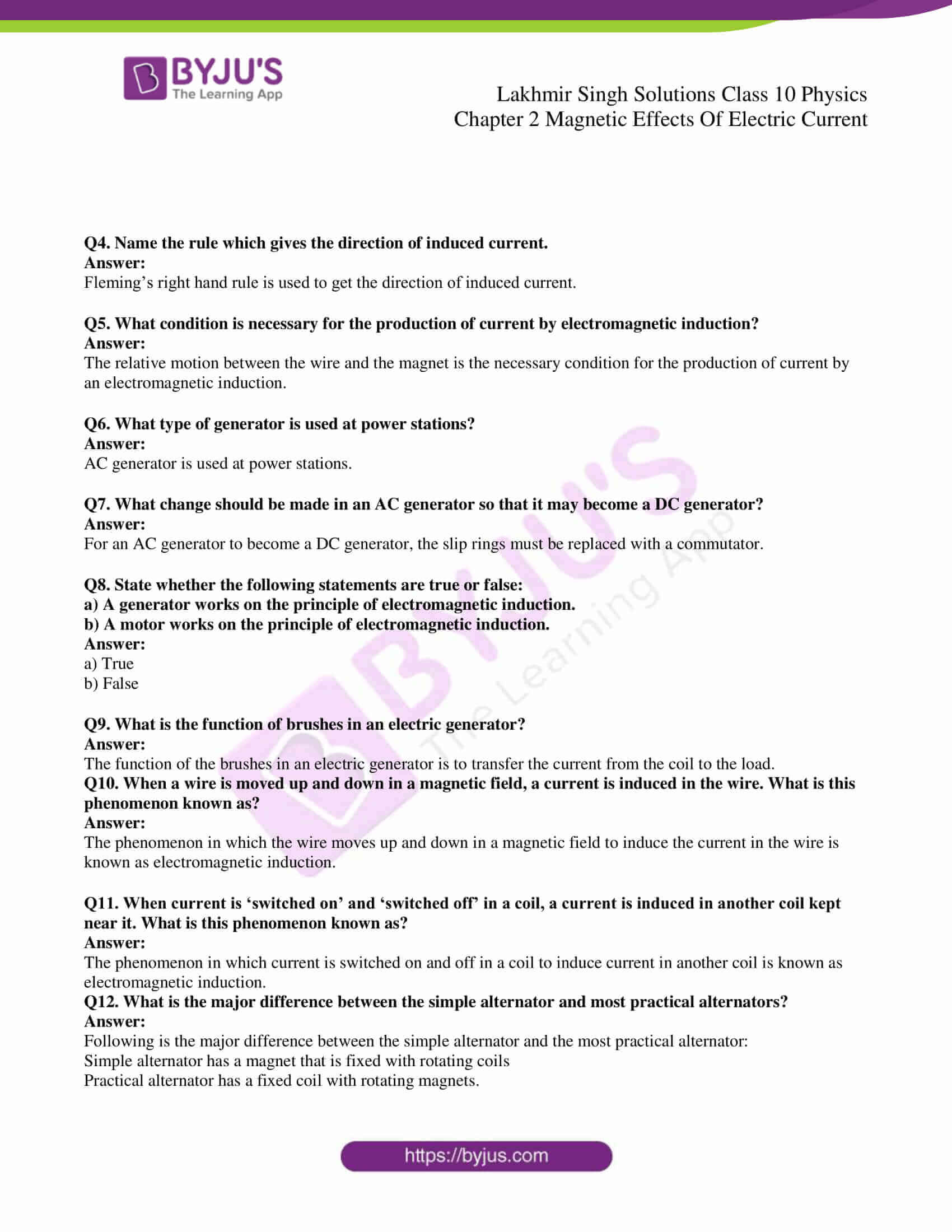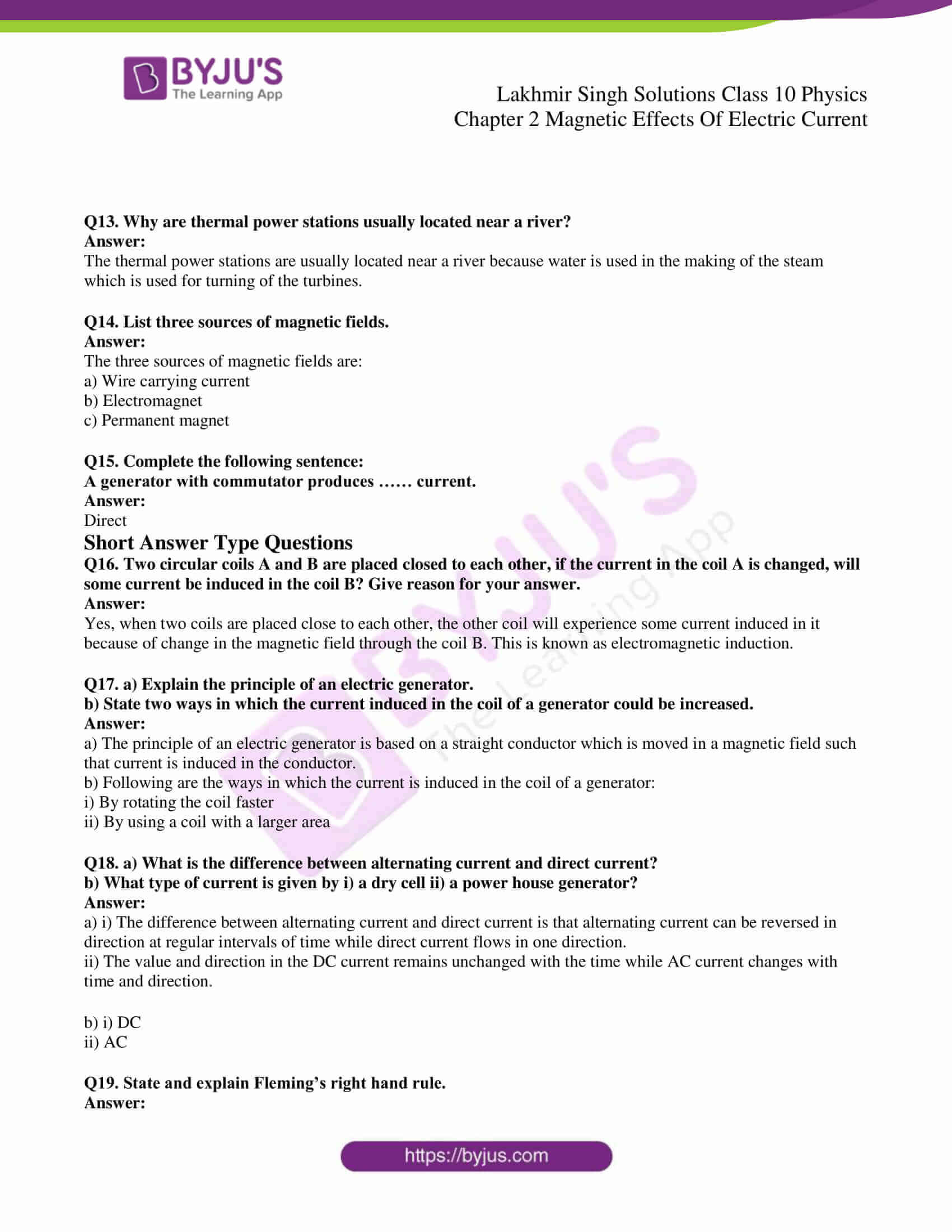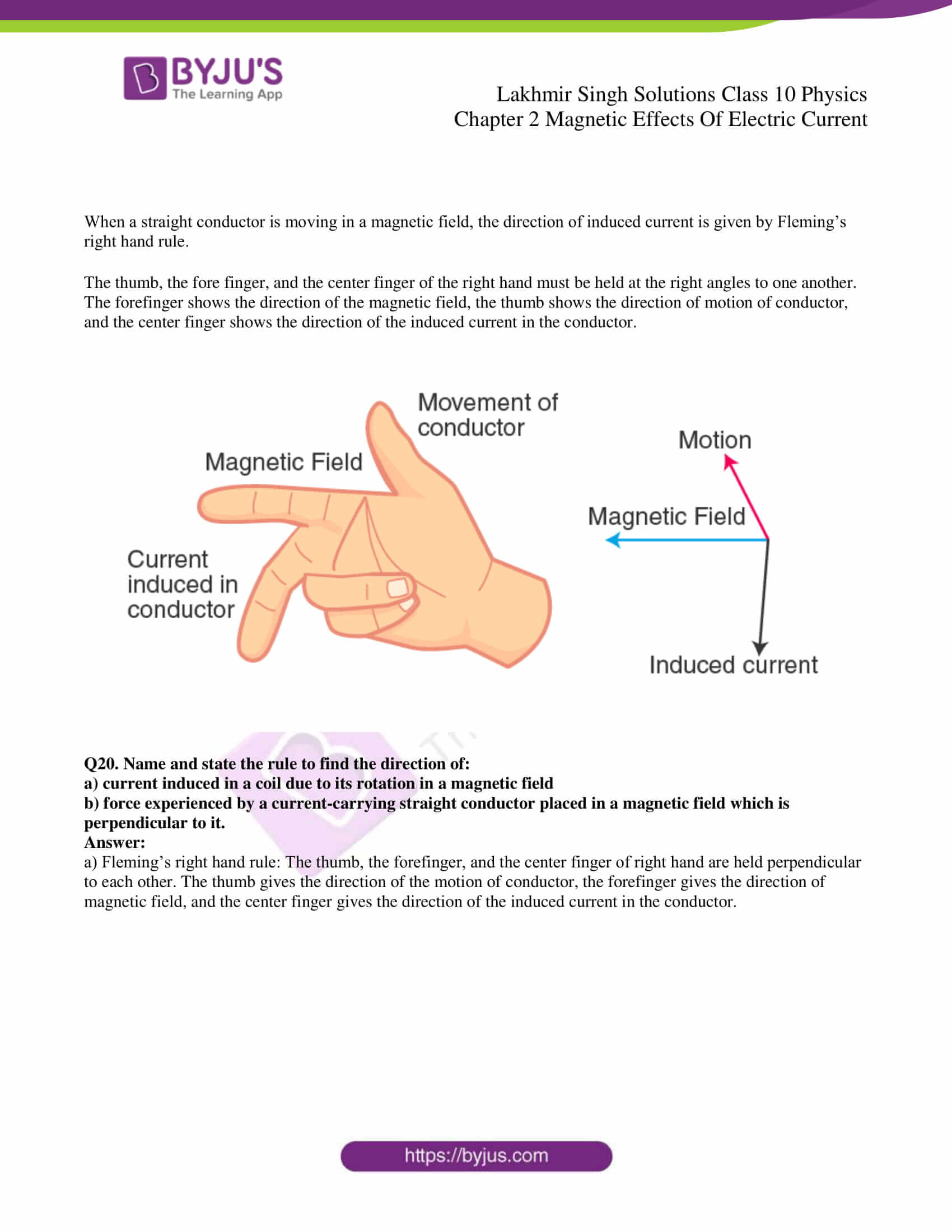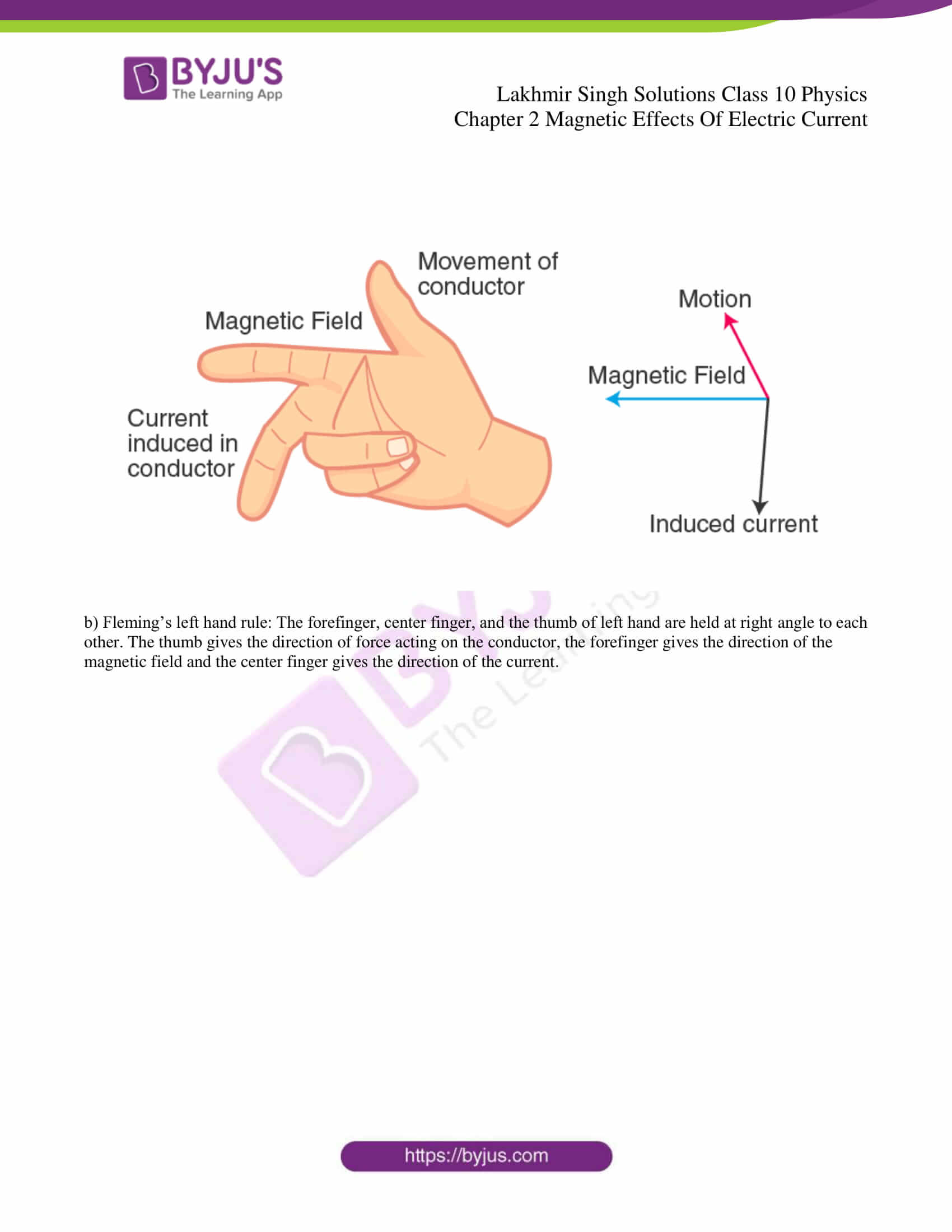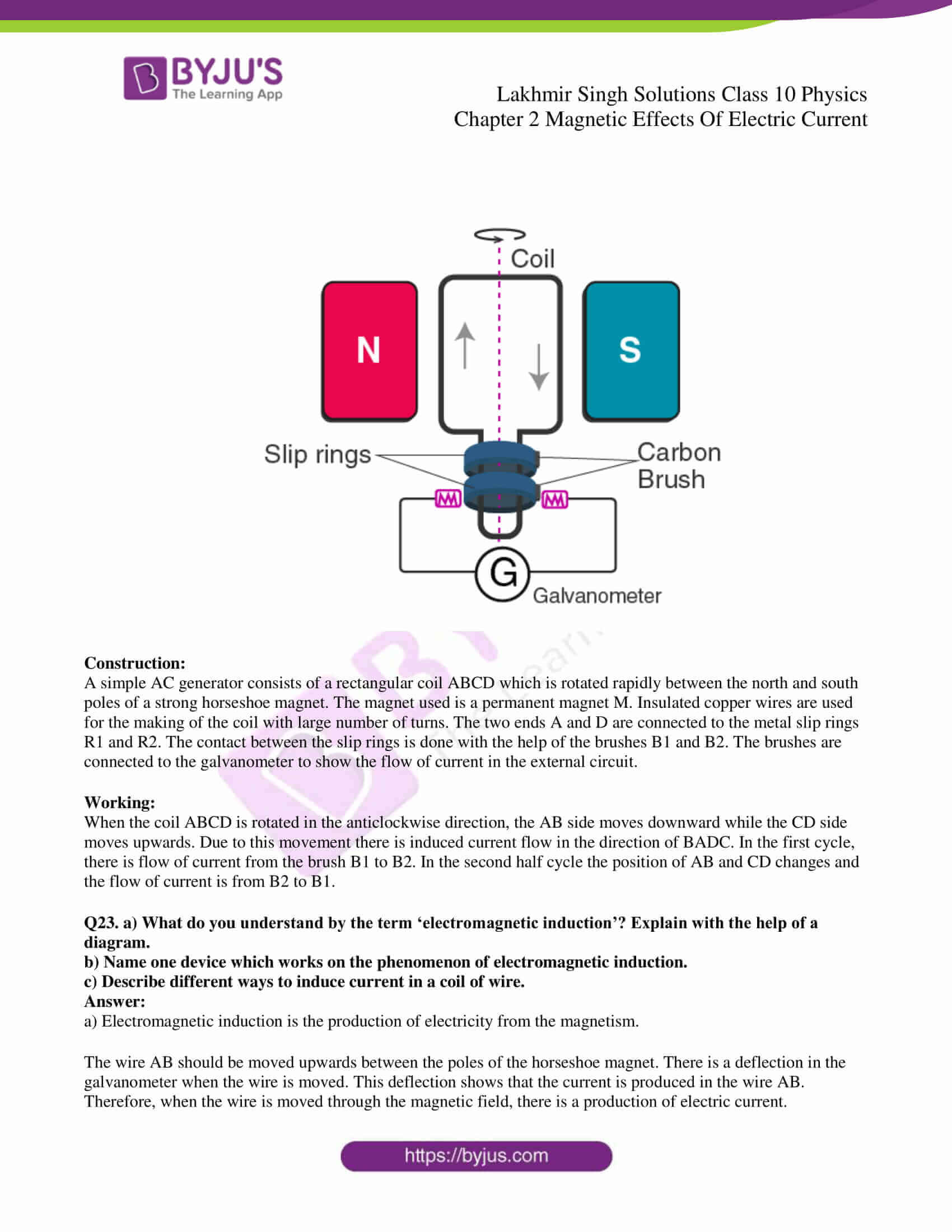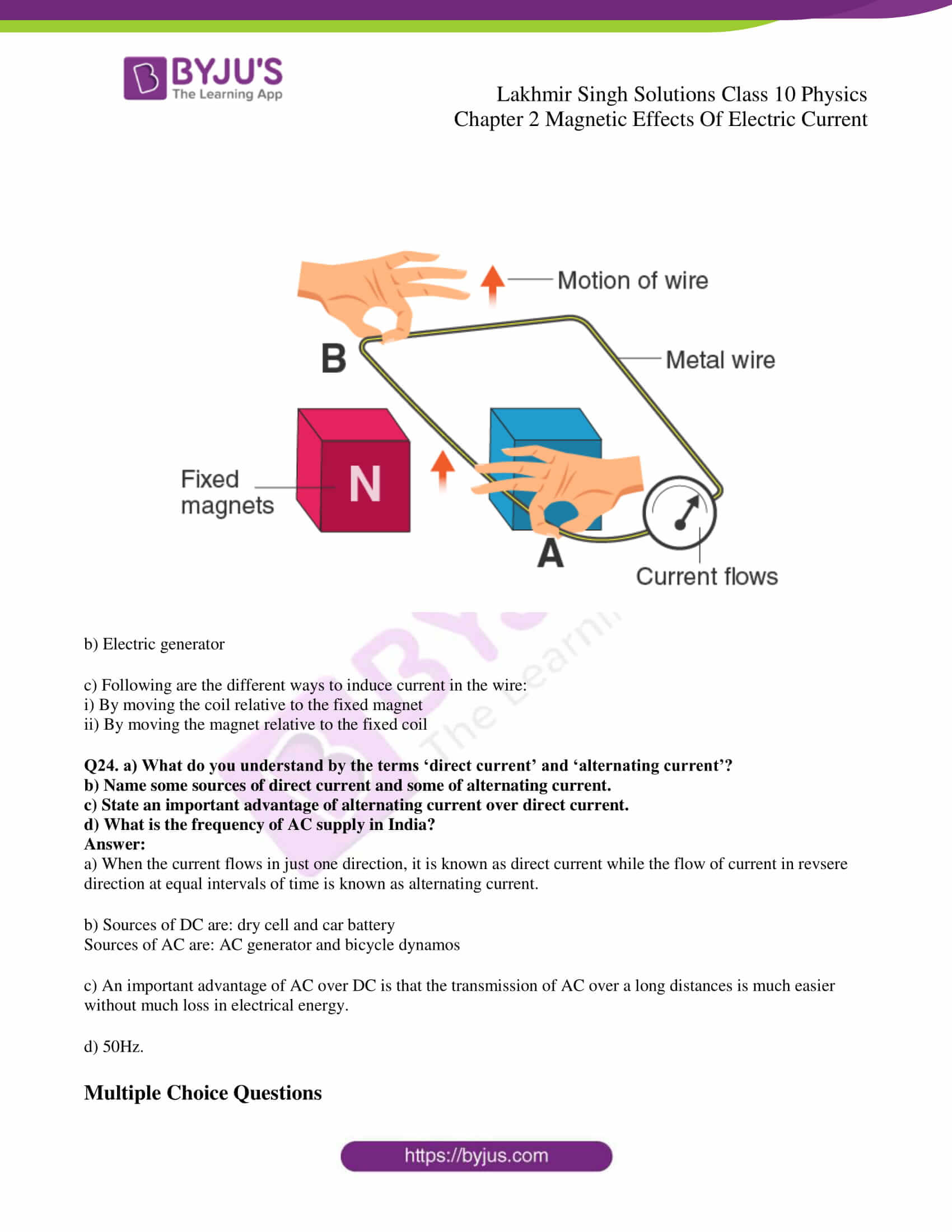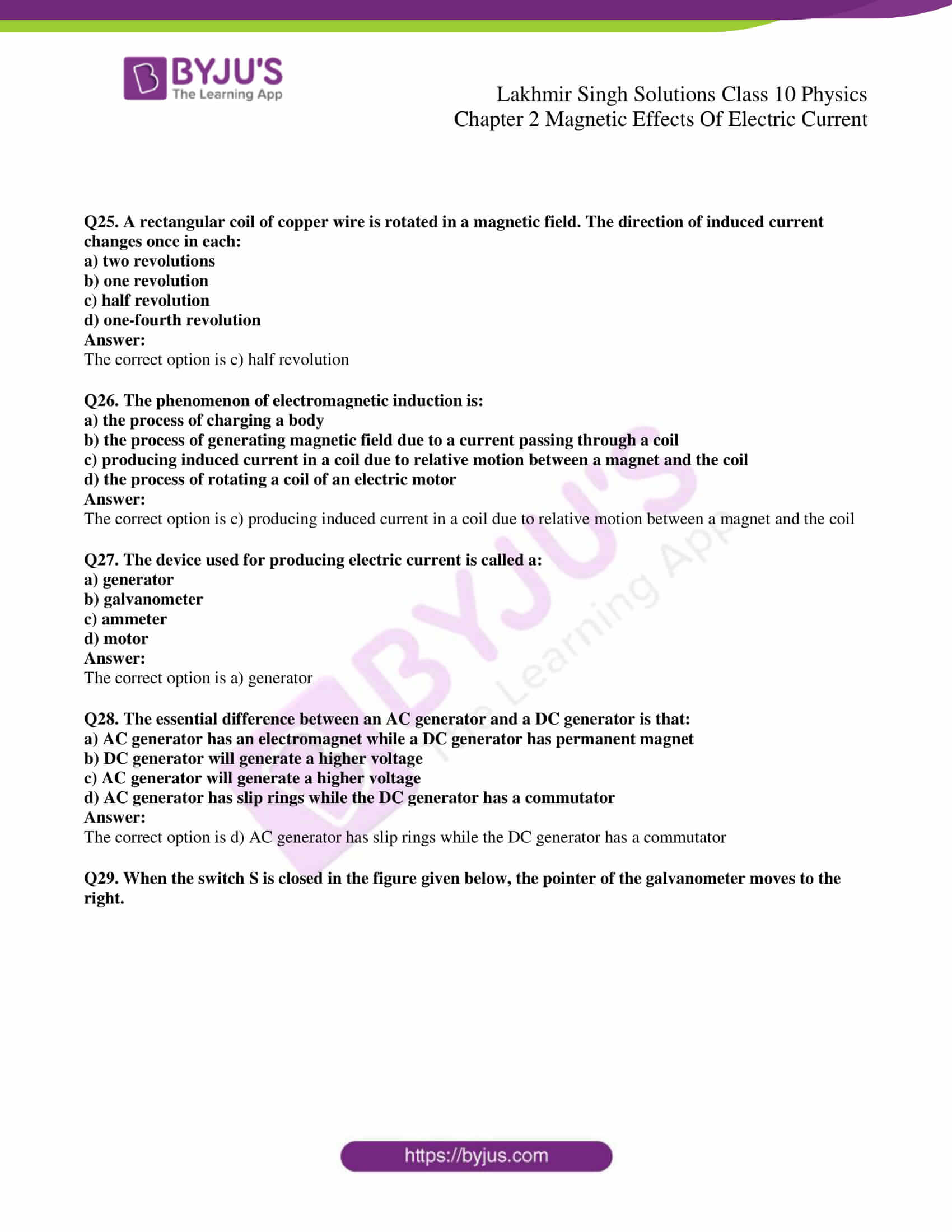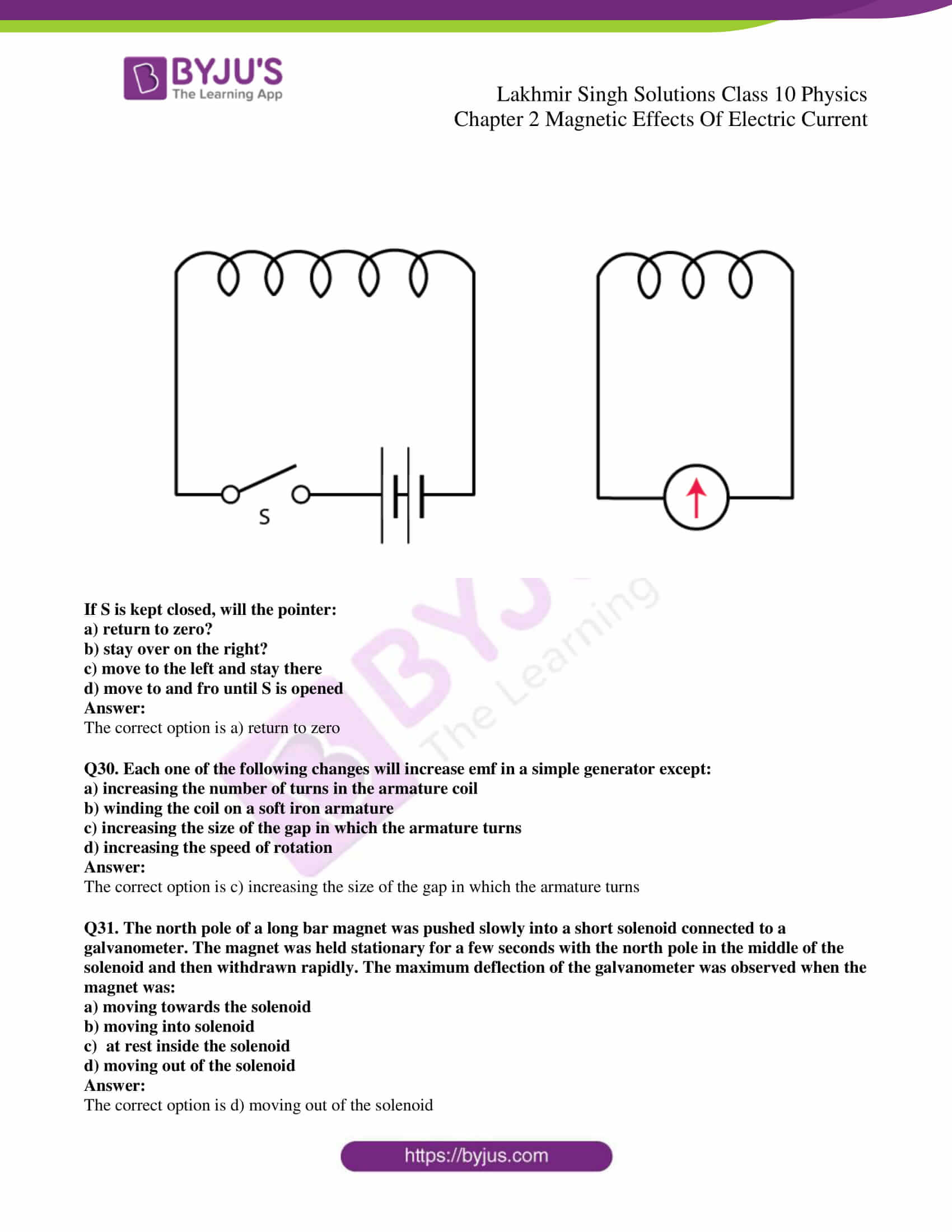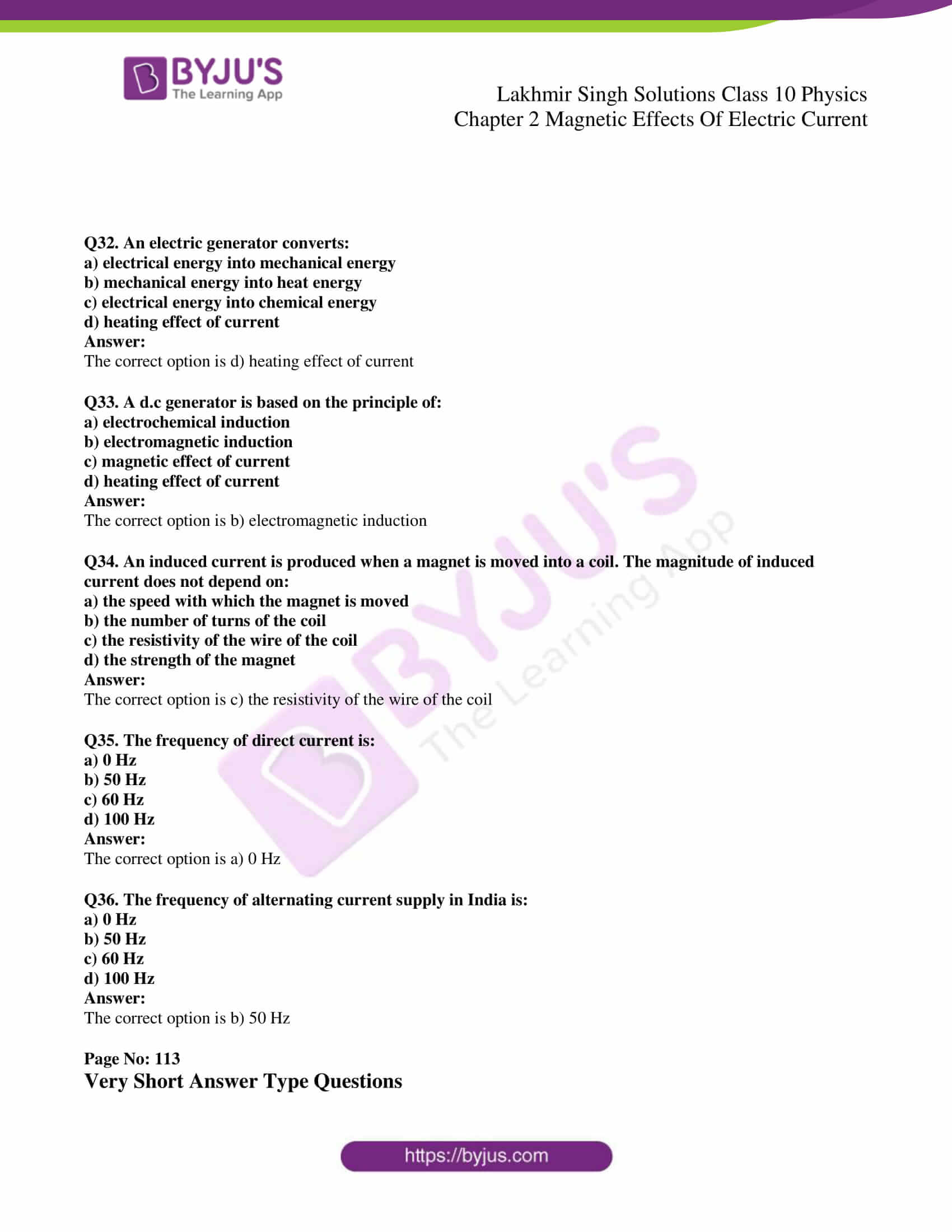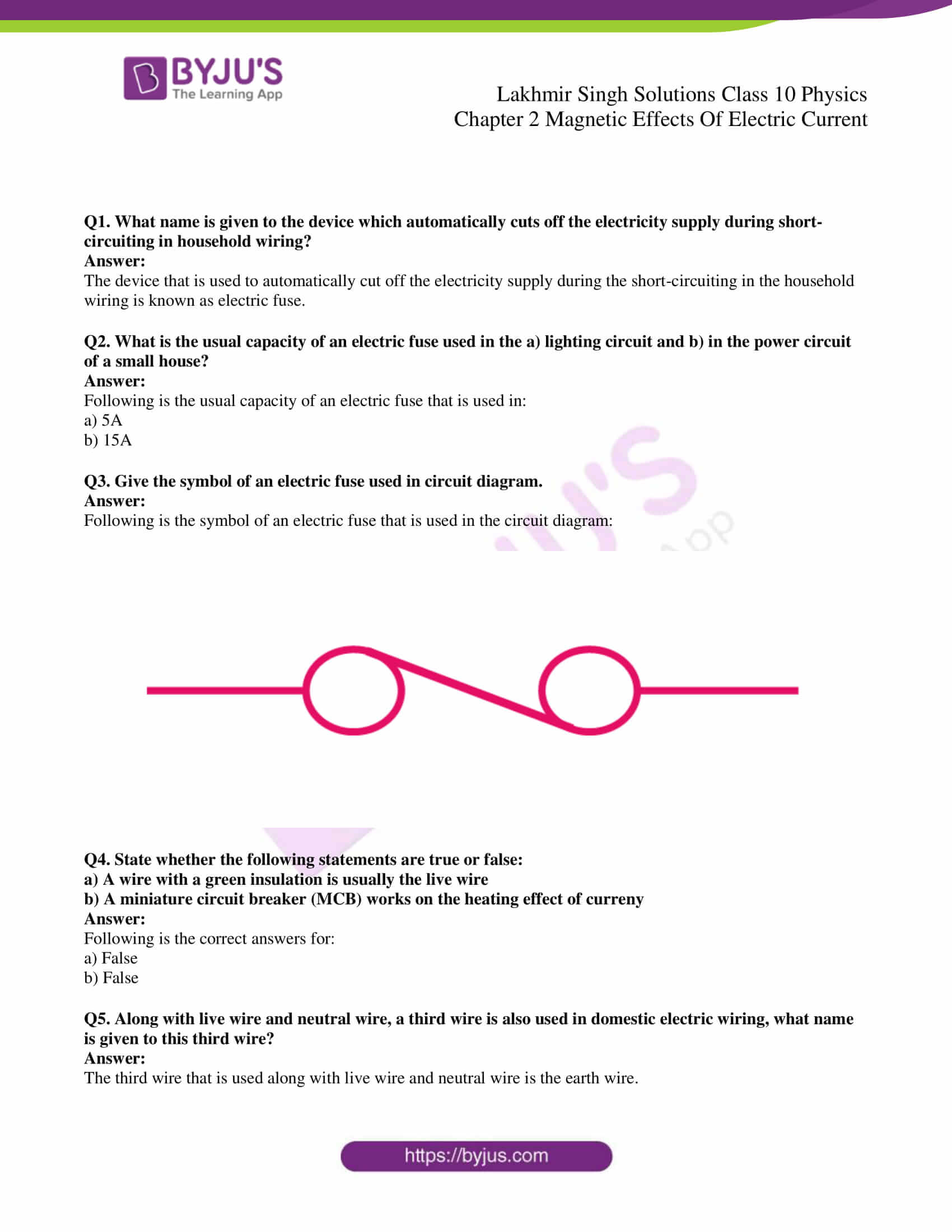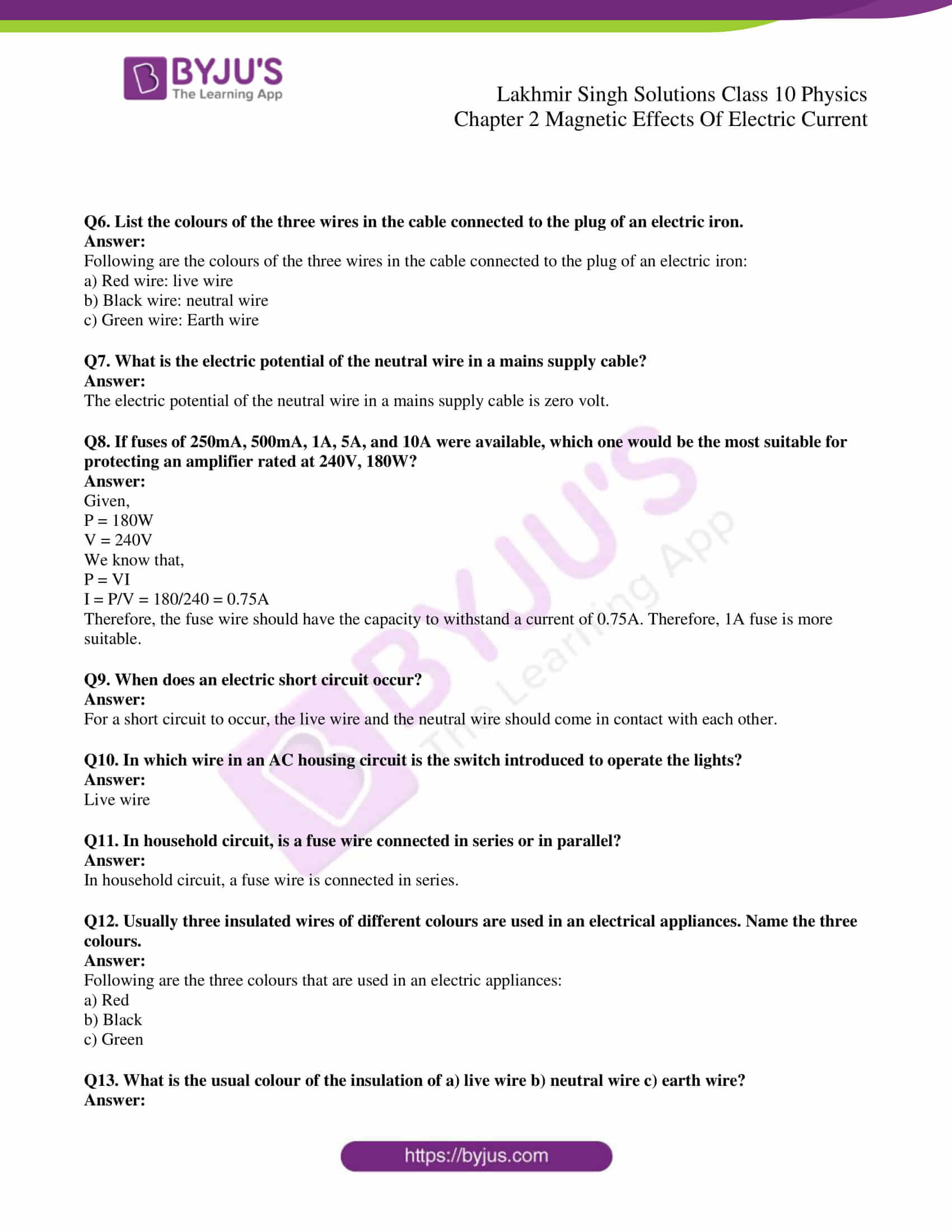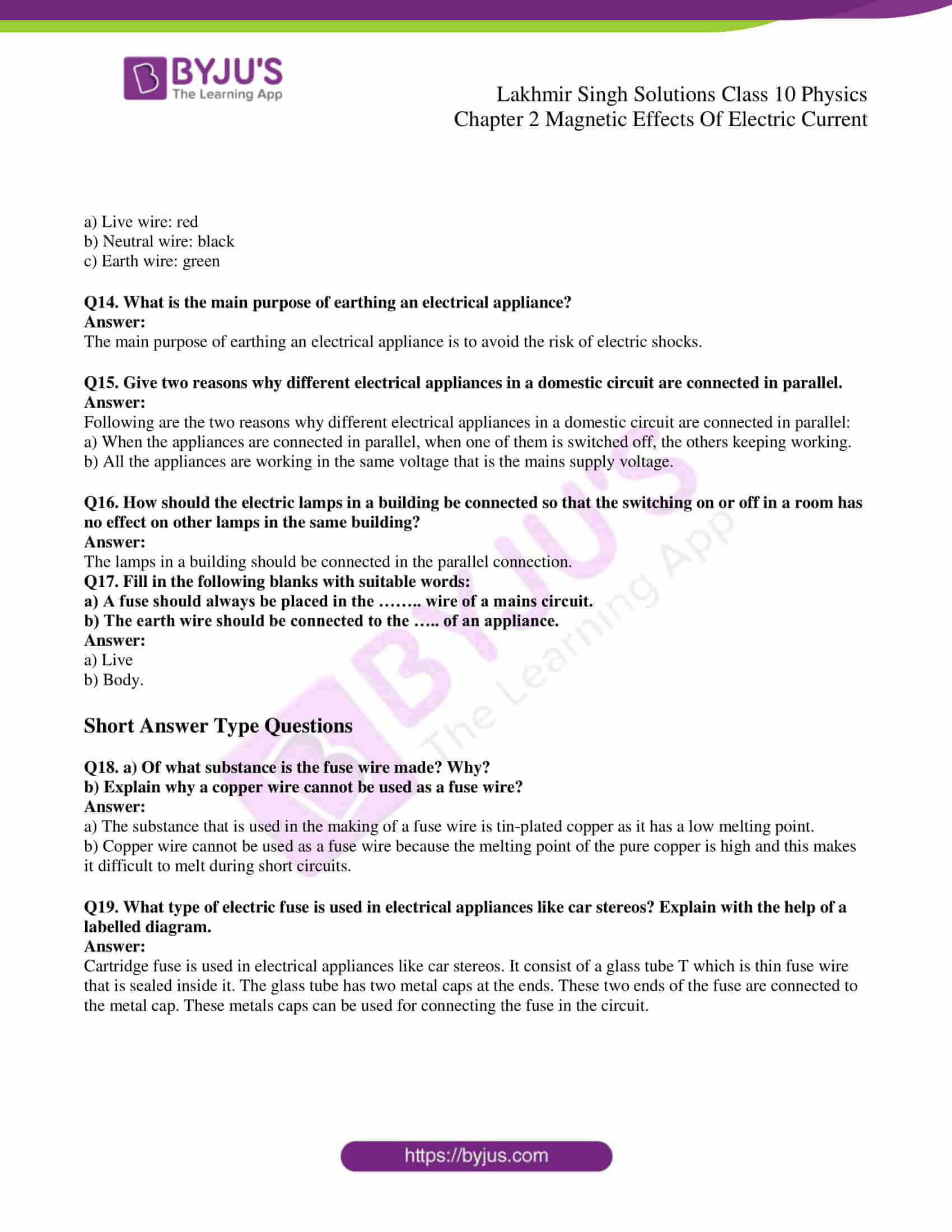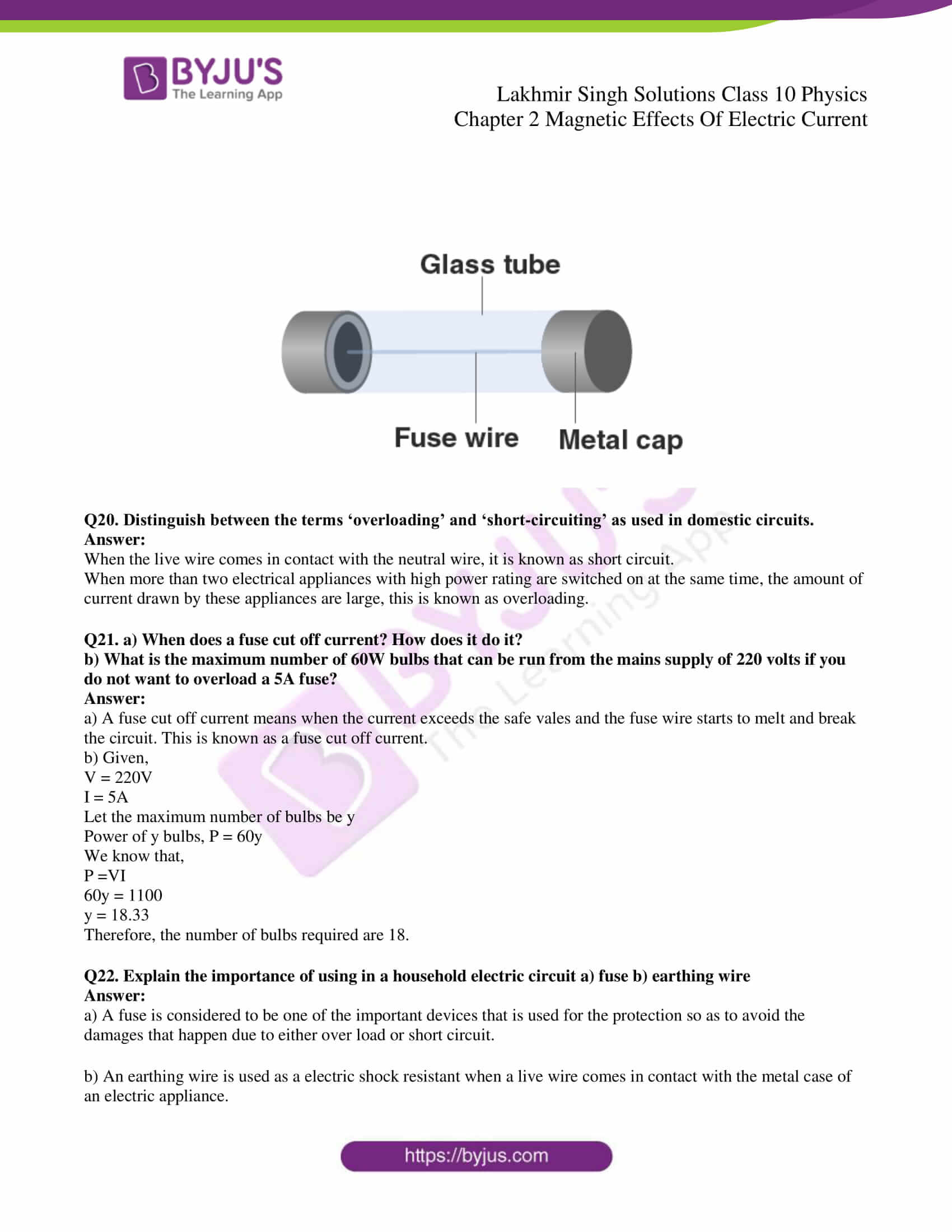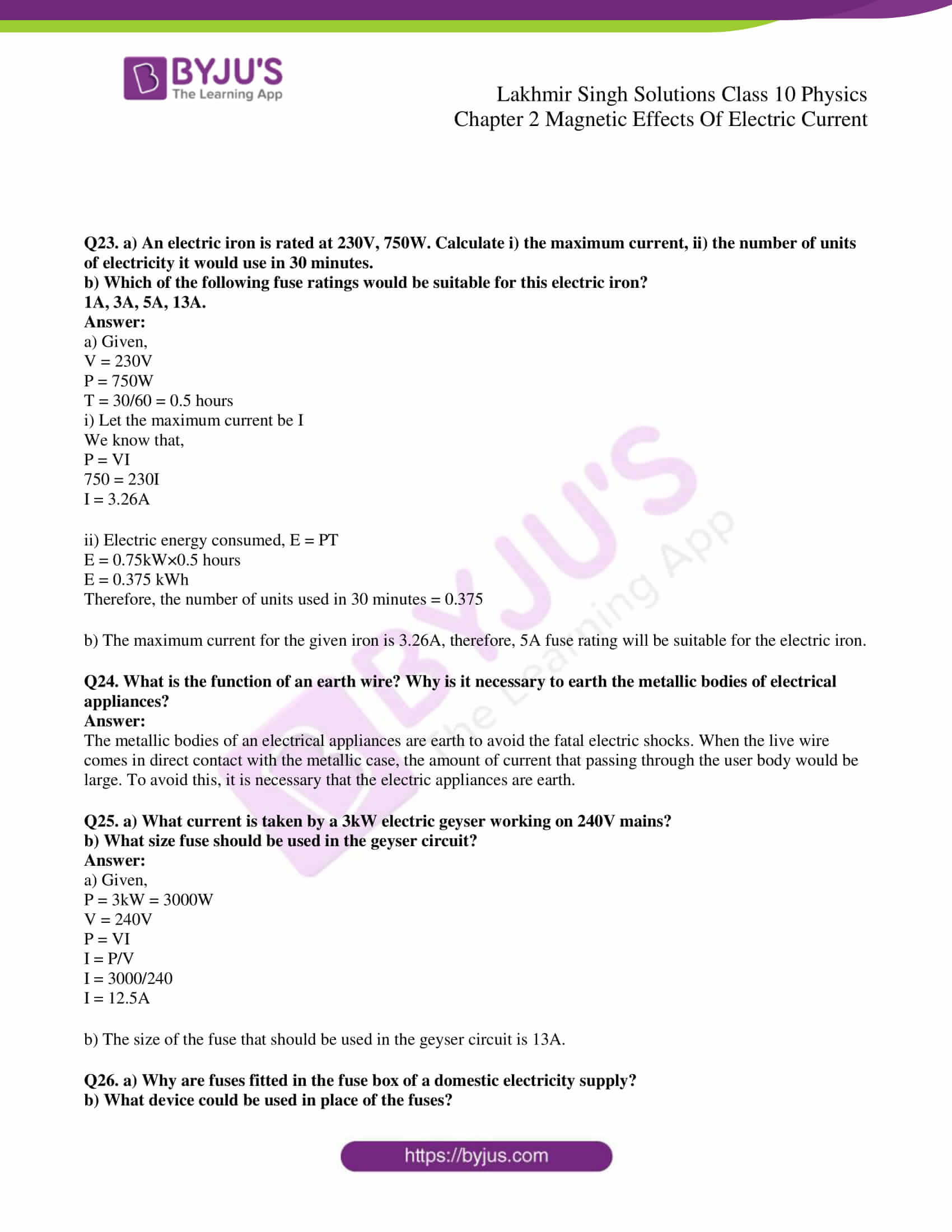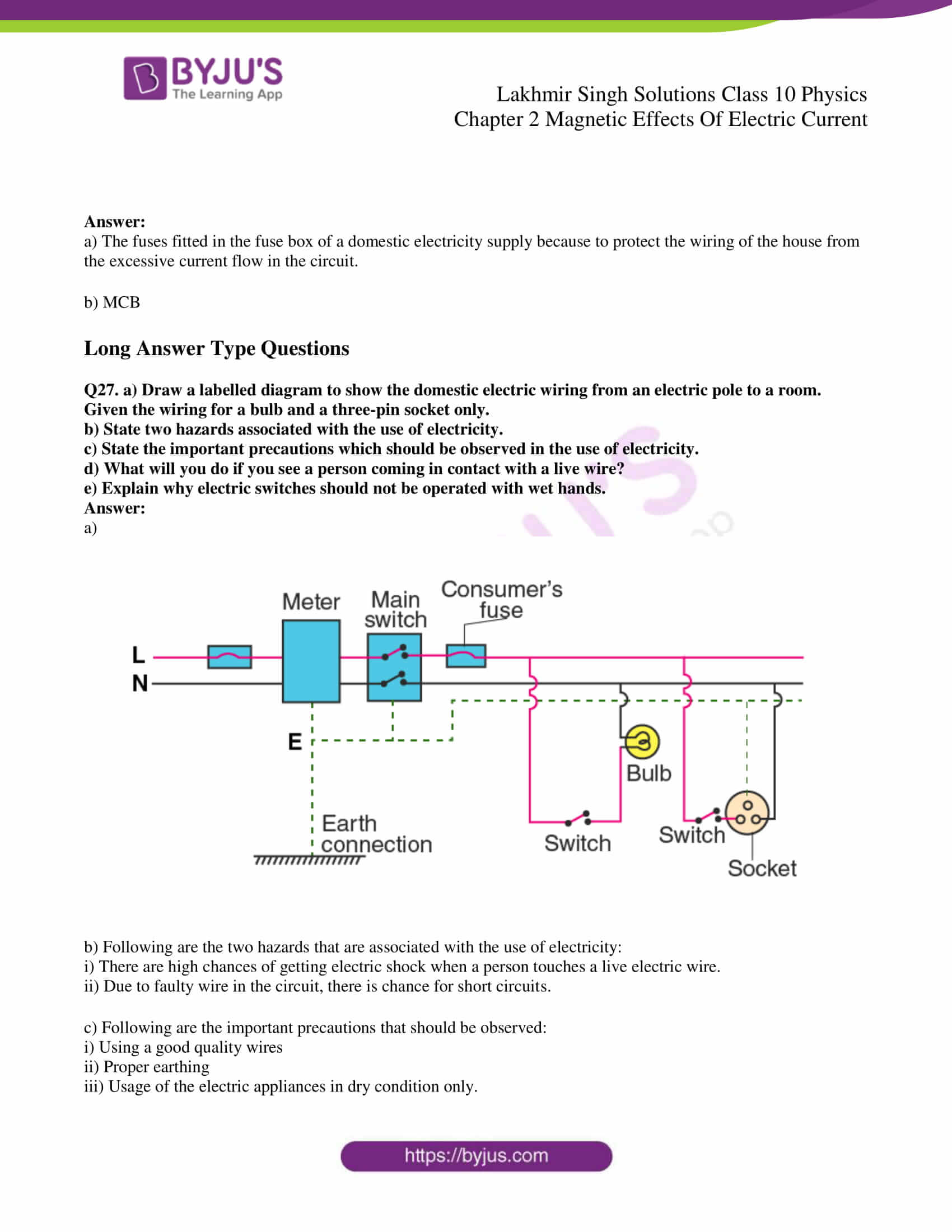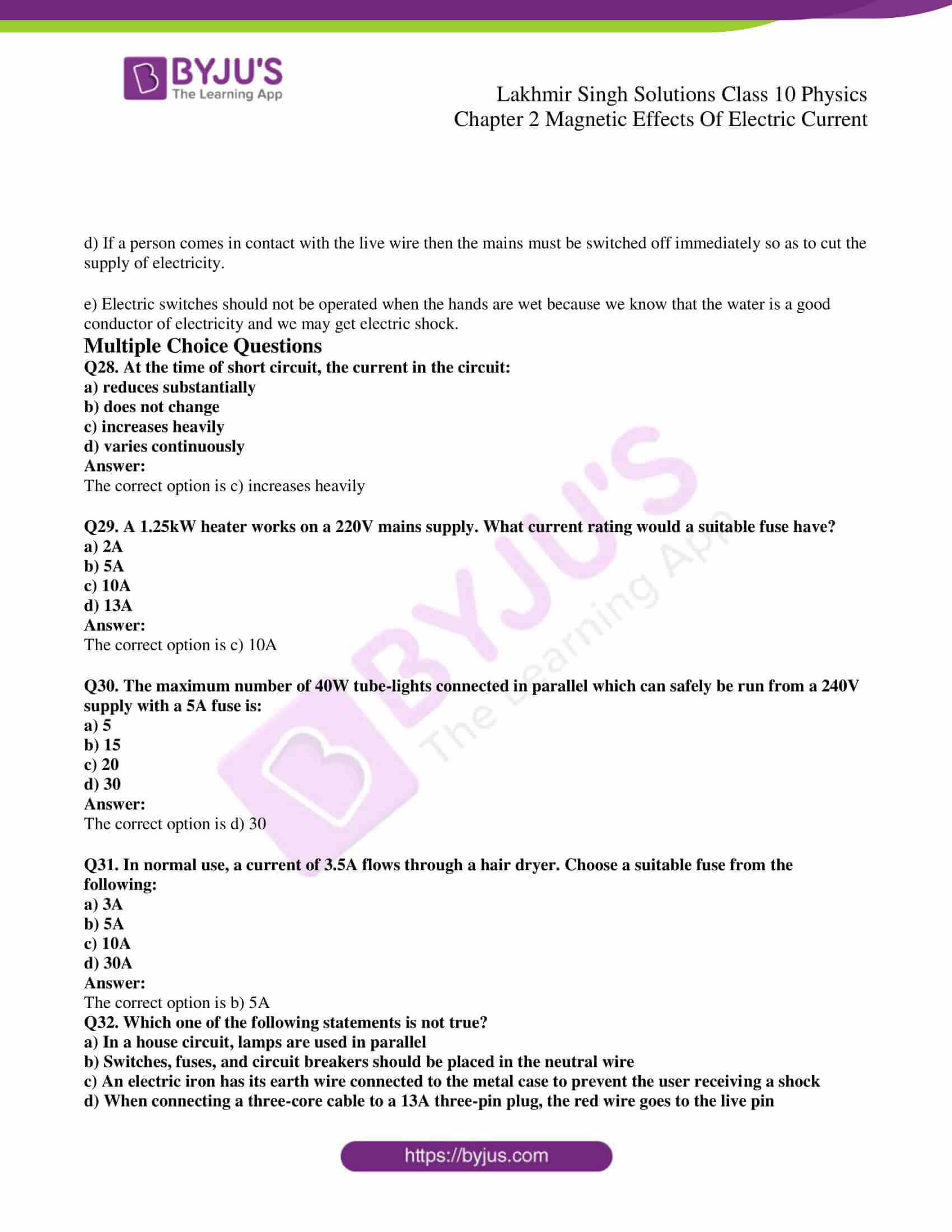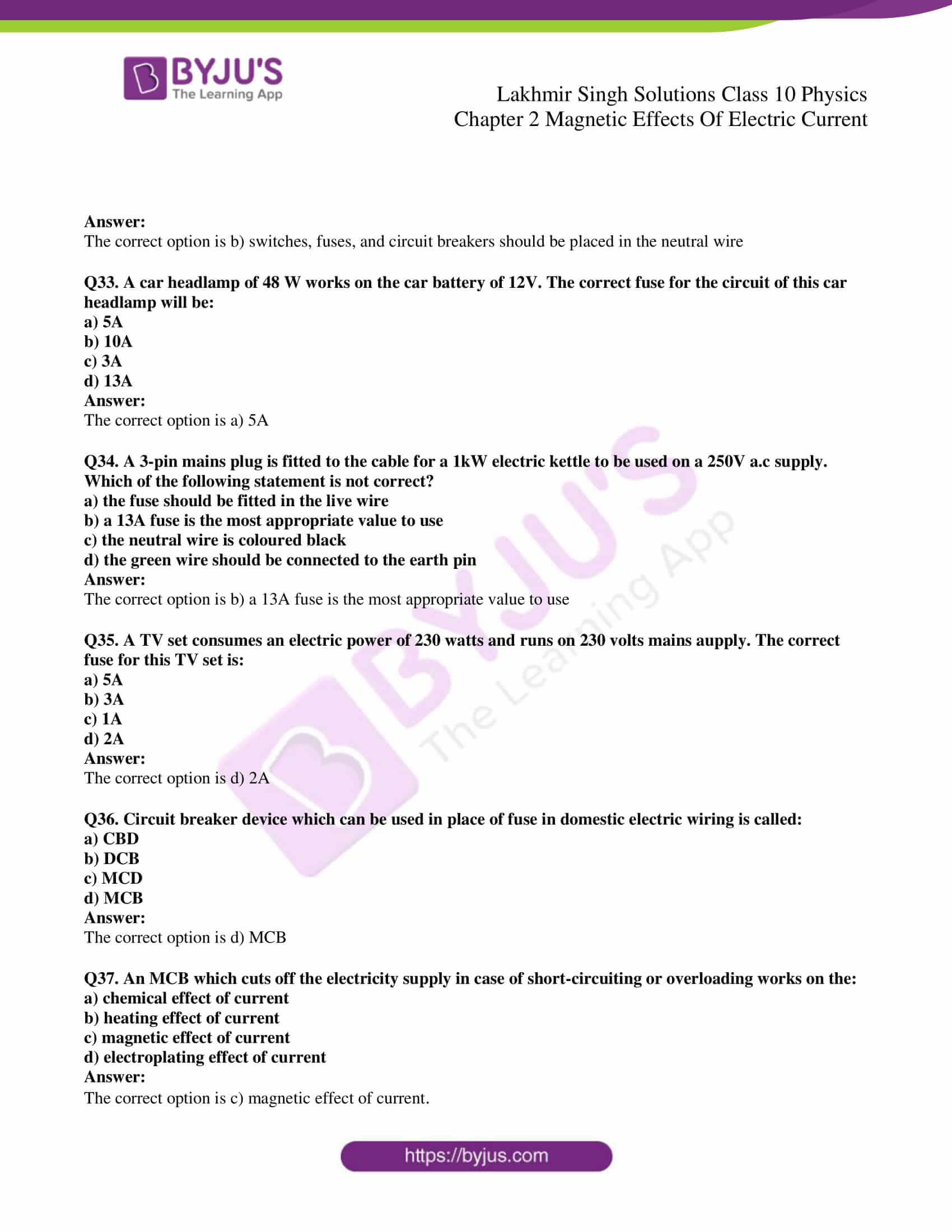### Access Lakhmir Singh Physics Class 10 Solutions For Chapter 2

Page No: 73

Q1. State any two properties of magnetic field lines.

Following are the properties of magnetic field lines:

a) The magnetic field lines always originate from the north pole and end in the south pole.

b) The magnetic field lines never cross each other.

Q2. What are the two ways in which you can trace the magnetic field pattern of a bar magnet?

Following are the two ways in which the magnetic field patter of a bar magnet can be traced:

a) By using iron filings

b) By using compass

Q3. You are given the magnetic field pattern of a magnet. How will you find out from it where the magnetic field is the strongest?

The magnetic field is the strongest when the magnetic field lines are closest to each other.

Q4. State whether the following statement is true or false:

The axis of earth’s imaginary magnet and the geographical axis coincide with each other.

False

The axis of earth’s imaginary magnet and the geographical axis are inclined to each other 15 degrees.

Q5. Why does a compass needle get deflected when brought near a bar magnet?

A compass needle gets deflected when brought near a bar magnet because the needle experiences a magnetic field exerted by the bar magnet.

Q6. Where do the manufacturers use a magnetic strip in the refrigerator? Why is the magnetic strip used?

Magnetic strips are used in the doors of the refrigerator to keep the door closed.

Q7. Fill in the following blanks with suitable words:

a) Magnetic field lines leaves the …. Pole of a bar magnet and enters at its …..

b) The earth’s magnetic field is rather like that of a ….. magnet with its …. Pole in the northern hemisphere.

a) North and south

b) Bar and south

Q8. Draw a diagram to show the magnetic field lines around a bar magnet.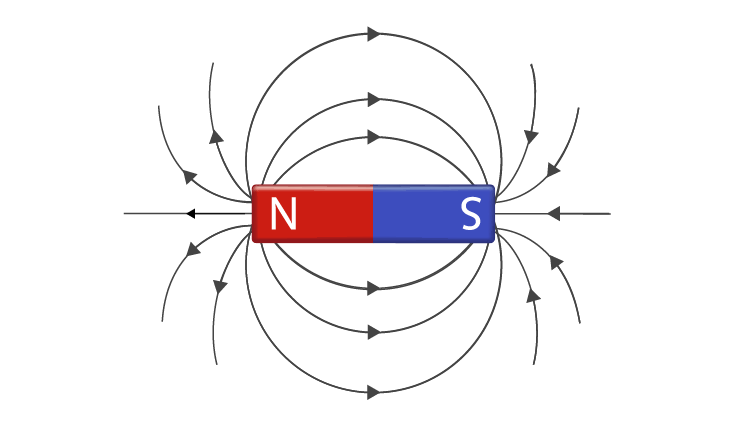Q9. What is a magnetic field? How can the direction of magnetic field lines at a place be determined?

Magnetic field is the space that surrounds the magnet in which the magnetic force is exerted. The direction of the magnetic field lines at a place can be determined by using a compass needle. The needle of the compass gets deflected when it placed near the magnetic field. The north end of the compass indicates the direction of magnetic field.

Q10. Explain why two magnetic field lines do not intersect each other?

The two magnetic field lines do not intersect each other because the resultant force on the north pole will always be in one direction. When the magnetic field lines intersect, the resultant force will in two directions which is not possible.

Q11. When an electric current is passed through any wire, a magnetic field is produced around it. Then why an electric iron connecting cable does not attract iron objects when electric current is switched on through it?

As the strength of the magnetic field produced by the cable is weak.

Q12. a) Define magnetic field lines. Describe an activity to draw a magnetic field line outside a bar magnet from one pole to another pole.

b) Explain why a freely suspended magnet always points in the north-south direction.

a) There will be a movement in the magnetic field lines when the north magnetic pole is moved. The magnetic field lines are also known as magnetic lines of force.

Activity:

Take a small compass and a bar magnet.

Place the bar magnet on a fixed placed on the drawing board using a adhesive material.

The boundary of the magnet should be marked.

The compass should be placed near the north pole of the magnet. The south pole of the needle points towards the north pole of the magnet.

Mark the ends of the needle.

By moving the needle, we can see that the north pole is occupied by the south pole of the needle.

By joining the points marked, a smooth curve is obtained.

b) A freely suspended magnet points towards the north-south direction because the earth behave as a magnet with its south pole in the geographical north and the north pole in its geographical south.

Multiple Choice Questions

Q13. A strong bar magnet is placed vertically above a horizontal wooden board. The magnetic lines of force will be:

a) only in horizontal plane around the magnet

b) only in vertical plane around the magnet

c) in horizontal as well as in vertical planes around the magnet

d) in all the planes around the magnet

The correct option is d) in all the planes around the magnet

Q14. The magnetic field lines produced by a bar magnet:

a) originate from the south pole and end at its north pole

b) originate from the north pole and end at its east pole

c) originate from the north pole and end at its south pole

d) originate from the south pole and end at its west pole

The correct option is c) originate from the north pole and end at its south pole

Q15. Which of the following is not attracted by a magnet?

a) steel

b) cobalt

c) brass

d) nickel

The correct option is c) brass

Q16. The magnetic field lines:

a) intersect at right angles to one another

b) intersect at an angle of 45 to each other

c) do not cross one another

d) cross at an angle of 60 to one another

The correct option is c) do not cross one another

Q17. The north pole of earth’s magnet is in the:

a) geographical south

b) geographical east

c) geographical west

d) geographical north

The correct option is a) geographical south

Q18. The axis of earth’s magnetic field is inclined with the geographical axis at an angle of about:

a) 5

b) 15

c) 25

d) 35

The correct option is b) 15

Q19. The shape of the earth’s magnetic field resembles that of an imaginary:

a) U-shaped magnet

b) straight conductor carrying current

c) current-carrying circular coil

d) bar magnet

The correct option is d) bar magnet

Q20. A magnet attracts:

a) plastics

b) any metal

c) aluminum

d) iron and steel

The correct option is d) iron and steel

Q21. A plotting compass is placed near the south pole of a bar magnet. The pointer of plotting compass will:

a) point away from the south pole

b) point parallel to the south pole

c) point towards the south pole

d) point at right angles to the south pole

The correct option is c) point towards the south pole

Q22. The metallic pointer of a plotting compass gets deflected only when it is placed near a bar magnet because the pointer has:

a) electromagnetism

b) permanent magnetism

c) induced magnetism

d) ferromagnetism

The correct option is b) permanent magnetism

Q23. Which of the following statements is incorrect regarding magnetic field lines?

a) the direction of magnetic field at a point is taken to be the direction in which the north pole of a magnetic compass needle points

b) magnetic field lines are closed curves

c) if magnetic field lines are parallel and equidistant, they represent zero field strength

d) relative strength of magnetic field is shown by the degree of closeness of the field lines

The correct option is c) if magnetic field lines are parallel and equidistant, they represent zero field strength

Page No: 81

Q1. Which effect of current can be utilized in detecting a current-carrying wire concealed in a wall?

Magnetic effect of current can be utilized in detecting a current-carrying wire that is concealed in a wall.

Q2. What conclusion do you get from the observation that a current-carrying wire deflects a compass needle placed near it?

The conclusion that is drawn by observing a current-carrying wire that deflects a compass needle is that there is a production of magnetic field around the needle.

Q3. Name the scientist who discovered the magnetic effect of current.

Oersted was the scientist who discovered the magnetic effect of current.

Q4. State qualitatively the effect of inserting an iron core into a current-carrying solenoid.

When an iron core is inserted into a current-carrying solenoid, the magnetic field becomes very strong.

Q5. Name the rule for finding the direction of magnetic field produced by a straight current-carrying conductor.

The rule for finding the direction of magnetic field produced by a straight current-carrying conductor is Maxwell’s right hand thumb rule.

Q6. State the form of magnetic field lines around a straight current-carrying conductor.

The magnetic lines around a straight current-carrying conductor form a concentric circles.

Q7. What is the other name of Maxwell’s right-hand thumb rule?

The other name of Maxwell’s right-hand thumb rule is Maxwell’s corkscrew rule.

Q8. State whether the following statement is true or false:

The magnetic field inside a long circular coil carrying current will be parallel straight lines.

True.

Q9. What is the shape of a current-carrying conductor whose magnetic field pattern resembles that of a bar magnet?

Solenoid is the shape of a current-carrying conductor.

Q10. State three ways in which the strength of an electromagnet can be increased.

Following are the three ways in which the strength of an electromagnet can be increased:

a) By increasing the number of turns in the coil

b) By increasing the current flowing through the coil

c) By reducing the length of the air gap present between the poles

Q11. Fill in the following blanks with suitable words:

a) The lines of ….. round a straight current-carrying conductor are in the shape of …..

b) For a current-carrying solenoid, the magnetic field is like that of a ……..

c) The magnetic effect of a coil can be increased by increasing the number of ………, increasing the …….., or inserting an …. core.

d) If a coil is viewed from one end and the current flows in an anticlockwise direction, then this end is a pole.

e) If a coil is viewed from one end, and the current flows in a clockwise direction, then this end is a …..

a) Magnetic field and concentric circles

b) Bar magnet

c) Turns, current, and iron

d) North

e) South

Q12. Describe how you will locate a current-carrying wire concealed in a wall.

A current-carrying wire concealed in a wall can be located with the help of magnetic effect of the current by using a plotting compass. As the plotting compass is moved across the wall, a deflection is observed on the needle at the place where current-carrying wire is concealed.

Q13. Describe some experiment to show that the magnetic field is associated with an electric current.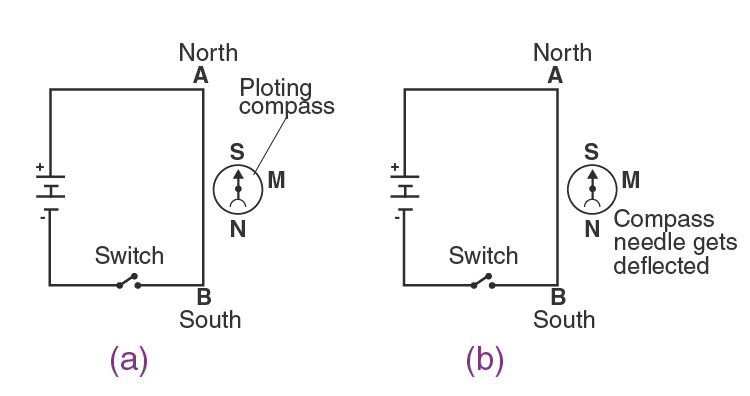A thick insulated copper wire AB is placed in the north-south direction as shown in the figure. A plotting compass M is placed under the copper wire AB. Both the ends of the wire are connected to the battery with the help of a switch. The compass needle is parallel to AB when there is no flow of current. As the current flows through the wire AB, a small deflection is seen in the needle. Therefore, the deflection in the needle is used for detecting the magnetic field associated with an electric current.

Q14. a) Draw a sketch to show the magnetic lines of force due to a current-carrying straight conductor.

b) Name and state the rule to determine the direction of magnetic field around a straight current-carrying conductor.

a)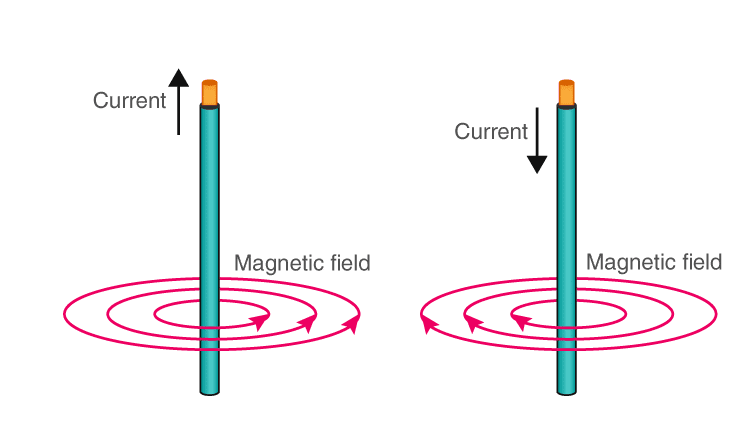b) The rule that is used to for determining the direction of magnetic field around a straight current-carrying conductor is Maxwell’s right hand rule. According to the rule, the thumb points at the direction of the current and the direction in which the fingers have encircled the current-carrying conductor gives the direction of the magnetic field lines around the wire.

Q15. State and explain Maxwell’s right hand thumb rule.

According to Maxwell’s right hand thumb rule, when a current-carrying conductor is held in the right hand, the thumb gives the direction of current and the fingers that have encircled the conductor gives the direction of the magnetic field lines around the wire.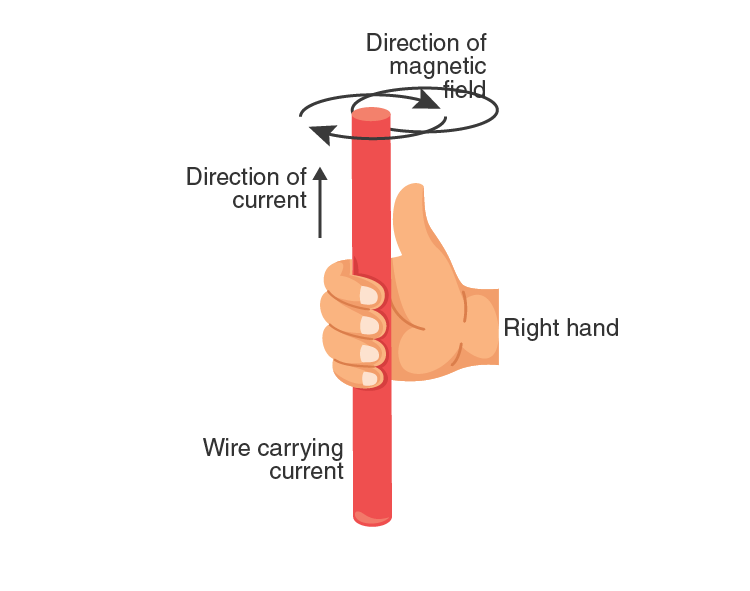Consider a straight current-carrying wire which is held vertically in the upward direction from A to B. The wire is held as in the given figure to find the direction of the magnetic field lines that is produced when the current flows through the wire. The thumb gives the direction of the current. The fingers that are encircled around the wire gives the direction of the magnetic field lines. The direction of the magnetic field lines is in the anticlockwise direction.

Q16. What is Maxwell’s corkscrew rule? For what purpose is it used?

According to the Maxwell’s corkscrew rule, the corkscrew is in the direction of the current and the handle gives the direction of the magnetic field. This rule is used for determining the direction of the magnetic field around the straight current-carrying conductor.

Q17. a) Draw the magnetic lines of force due to a circular wire carrying current.

b) What are the various ways in which the strength of magnetic field produced by a current-carrying circular coil can be increased?

a)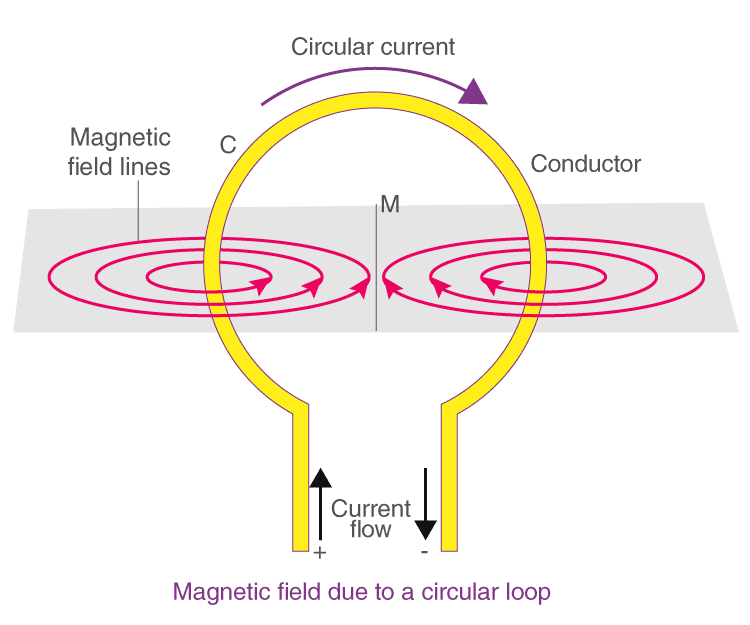b) The strength of the magnetic field produced by the current-carrying circular coil is increased by increasing the number of turns of the wire in the coil and by increasing the current flowing through the coil.

Q18. State and explain the clock face rule for determining the polarities of a circular wire carrying current.

According to the clock face rule, to look at one face of a circular wire when the current is passing through it is:

i) When the current around the face of the circular wire flows in the clockwise direction, then that face of the circular wire will be south pole.

ii) When the current around the face of the circular wire flows in the anti-clockwise direction, then that face of the circular wire will be north pole.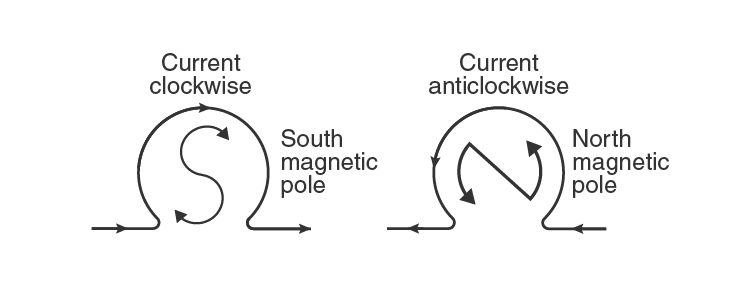Q19. Name any two factors on which the strength of magnetic field produced by a current-carrying solenoid depends. How does it depend on these factors?

The strength of the magnetic field produced by a current-carrying solenoid depends on:

a) the current flowing through the solenoid determines the strength of the solenoid

b) the number of turns in the solenoid determine the strength of the magnetic field in the solenoid.

Q20. a) Draw a circuit diagram to show how a soft iron piece can be transformed into an electromagnet.

b) Describe how an electromagnet could be used to separate copper from iron in a scrap yard.

a)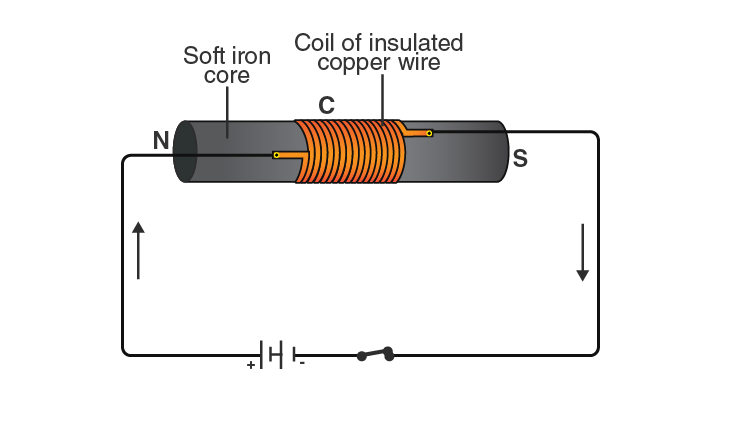An insulated copper wire is wound around the coil C such that the two ends of the copper wire are connected to a battery. Therefore, an electromagnet is used as a soft iron core.

b) To separate the copper from the iron in a scrap yard, electromagnetic cranes are used. The electromagnet is energized by making the current flow through it which helps in picking the iron pieces from the scrap yard.

Q21. a) How does an electromagnet differ from a permanent magnet?

b) Name two devices in which electromagnets are used and two devices where permanent magnets are used.

a) An electromagnet is different from a permanent magnet because electromagnet can produce the magnetic field as long as the current is flowing through it while a permanent magnet doesn’t require electric current for the production of magnetic field.

b) Electromagnets are electric bells and electric motors.

Permanent magnets are toys and refrigerator doors.

Q22. a) What is a solenoid? Draw a sketch to show the magnetic field pattern produced by a current-carrying solenoid.

b) Name the type of magnet with which the magnetic field pattern of a current-carrying solenoid resembles.

c) What is the shape of field lines inside a current-carrying solenoid? What does the pattern of filed lines inside a current-carrying solenoid indicate?

d) List three ways in which the magnetic field strength of a current-carrying solenoid can be increased?

e) What type of core should be put inside a current-carrying solenoid to make an electromagnet?

a) A solenoid is defined as a long coil which contains a large number of close turns of insulated copper wire.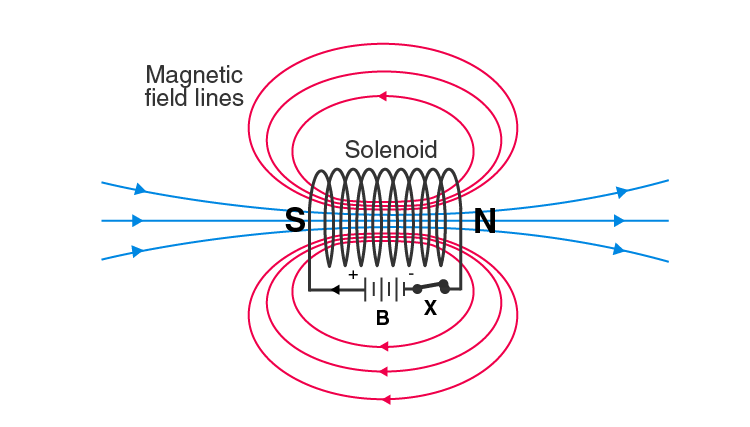b) The magnetic field produced by a solenoid and a bar magnet are similar to each other.

c) The magnetic field lines inside a current-carrying solenoid are parallel to each other and are in straight lines which means that the magnetic field inside a solenoid is uniform.

d) The magnetic field strength of a current-carrying solenoid can be increased by increasing the number of turns in the solenoid, by using a soft iron as a core, and by increasing the current flow through the solenoid.

e) Soft iron core

Q23. a) What is an electromagnet? Describe the construction and working of an electromagnet with the help of a labelled diagram.

b) Explain why an electromagnet is called a temporary magnet.

c) Explain why the core of an electromagnet should be of soft iron and not of steel.

d) State the factors on which the strength of an electromagnet depends. How does it depend on these factors?

e) Write some of the important uses of electromagnets.

a) An electromagnet is a temporary magnet which works when current is passed through it. To make an electromagnet, a soft iron is taken with an insulated copper wire wound to it. The two ends of the copper wire are connected to the battery for making an electromagnet.b) An electromagnet is also known as a temporary magnet because the magnetic field produced by an electromagnet is dependent on the flow of current through its coil.

c) The core of an electromagnet should be a soft iron and not a steel because soft iron loses its magnetism when there is no current flow through it while steel does not lose its magnetism in the absence of electric current.

d) The strength of an electromagnet depends on the number of turns of the coil, the length of the air gap between the poles, and the current flowing through the coil.

e) Electromagnets find applications in electrical devices such as in loudspeaker, electric bell, and electric motor. They are also used by doctors for treating their patient’s when a piece of iron is stuck in the wounds.

Multiple Choice Questions

Q24. The strength of the magnetic field between the poles of an electromagnet would be unchanged if:

a) current in the electromagnet winding were doubled

b) direction of current in electromagnet were doubled

c) distance between the poles of electromagnet were doubled

d) material of the core of electromagnet were changed

The correct option is b) direction of current in electromagnet were doubled

Q25. The diagram given below represents magnetic field caused by a current-carrying conductor which is: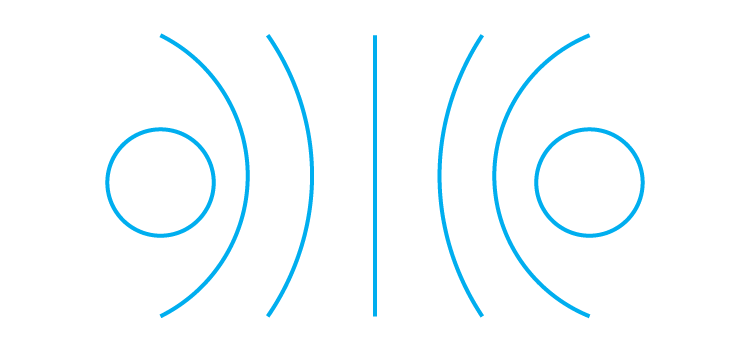a) a long straight wire

b) a circular coil

c) a solenoid

d) a short straight wire

The correct option is b) a circular coil

Q26. The magnetic field inside a long straight solenoid carrying current:

a) is zero

b) decreases as we move towards its ends

c) increases as we move towards its ends

d) is the same at all points

The correct option is d) is the same at all points

Q27. Which of the following correctly describes the magnetic field near a long straight wore?

a) the field consists of straight lines perpendicular to the wire

b) the field consists of straight lines parallel to the wire

c) the field consists of radial lines originating from the wire

d) the field consists of concentric circles centered on the wire

The correct option is d) the field consists of concentric circles centered on the wire

Q28. The north-south polarities of an electromagnet can be found easily by using:

a) Fleming’s right-hand rule

b) Fleming’s left-hand rule

c) Clock face rule

d) Left-hand thumb rule

The correct option is c) Clock face rule

Q29. The direction of current in the coil at one end of an electromagnet is clockwise. This end of the electromagnet will be:

a) north pole

b) east pole

c) south pole

d) west pole

The correct option is c) south pole

Q30. If the direction of electric current in a solenoid when viewed from a particular end is anticlockwise, then this end of solenoid will be:

a) west pole

b) south pole

c) north pole

d) east pole

The correct option is c) north pole

Q31. The most suitable material for making the core of an electromagnet is:

a) soft iron

b) brass

c) aluminum

d) steel

The correct option is a) soft iron

Q32. The magnetic effect of current was discovered by:

a) Maxwell

b) Fleming

c) Oersted

The correct option is c) Oersted

Q33. A soft iron bar is inserted inside a current-carrying solenoid. The magnetic field inside the solenoid:

a) will decrease

b) will increase

c) will become zero

d) will remain the same

The correct option is b) will increase

Q34. The magnetic field lines in the middle of the current-carrying solenoid are:

a) circles

b) spirals

c) parallel to the axis of the tube

d) perpendicular to the axis of the tube

The correct option is c) parallel to the axis of the tube

Q35. The front face of a circular wire carrying current behaves like a north pole. The direction of current in this face of the circular wire is:

a) clockwise

b) downwards

c) anticlockwise

d) upwards

The correct option is c) anticlockwise

Q36. The back face of a circular loop of wire is found to be south magnetic pole. the direction of current in this face of the circular loop of wire will be:

a) towards south

b) clockwise

c) anticlockwise

d) towards north

The correct option is b) clockwise

Page No: 91

Q1. What happens when a current-carrying conductor is placed in a magnetic field?

When a current-carrying conductor is placed in a magnetic field, a mechanical force is experienced by the conductor which is responsible for the movement of the conductor.

Q2. When is the force experience by a current-carrying conductor placed in a magnetic field largest?

The force experience by a current-carrying conductor when placed in a magnetic field is largest when the conductor is at right angle to the magnetic field.

Q3. In a statement of Fleming’s left hand rule, what do the following represent?

a) direction of center finger

b) direction of forefinger

c) direction of thumb

a) Current

b) Magnetic field

c) Force acting on the conductor

Q4. Name one device which works on the magnetic effect of current.

The device that works on the magnetic effect of current is an electric bell. It is used as an electromagnet to produce sound.

Q5. Name the device which converts electrical energy into mechanical energy.

Electric motor is the device which converts electric energy into mechanical energy.

Q6. A motor converts one form of energy into another. Name the two forms.

The two forms of energies that are involved are:

a) Electrical energy

b) Mechanical energy

Q7. State whether the following statement is true or false:

An electric motor converts mechanical energy into electrical energy.

The given statement is false.

An electric motor converts electrical energy into mechanical energy.

Q8. For Fleming’s left hand rule, write down the three things that are 90 to each other, and next to each one write down the finger or thumb that represents it.

a) Current: the direction is given by the center finger

b) Magnetic field: the direction is given by the fore finger

c) Force or motion: the direction is given the thumb.

Q9. Name the device which is used to reverse the direction of current in the coil of a motor.

For the reversal of the direction of current in the coil of a motor, a commutator is used.

Q10. What is the other name of the split ring used in an electric motor?

The other name of the split ring is commutator.

Q11. What is the function of a commutator in an electric motor?

The function of a commutator in an electric motor is to reverse the direction of the flow of the current through the coil whenever the coil passed the vertical position during the revolution.

Q12. Of what substance are the brushes of an electric motor made?

The brushes of an electric motor are made from the carbon.

Q13. Of what substance is the core of the coil of an electric motor made?

The core of the coil of an electric motor is made from the soft iron.

Q14. In an electric motor, which of the following remains fixed and which rotates with the coil?

Commutator and brush

Brush remains fixed while commutator rotates with the coil.

Q15. What is the role of the split ring in an electric motor?

The role of the split ring in an electric motor is to reverse the direction of current flow through the coil whenever the coil passes through the vertical position during the revolution.

Q16. Fill in the following blanks with suitable words:

a) Fleming’s rule for the motor effect uses the ……

b) A motor contains a kind of switch called a …. Which reverses the current every half …..

a) Left

c) Commutator and rotation

Q17. a) A current-carrying conductor is placed perpendicularly in a magnetic field. Name the rule which can be used to find the direction of force acting on the conductor.

b) State two ways to increase the force on a current-carrying conductor in a magnetic field.

c) Name one device whose working depends on the force exerted on a current-carrying coil placed in a magnetic field.

a) Fleming’s left hand rule

b) When the current flow in the conductor is increased, the strength of the magnetic field also increases.

c) Electric motor.

Q18. State Fleming’s left-hand rule. Explain it with the help of labelled diagrams.

Fleming’s left hand rule: Hold the forefinger, the center finger, and the thumb of the left hand at right angles to each other. The forefinger gives the direction of the force acting on the direction, the center finger gives the direction of current, and the forefinger gives the direction of the magnetic field.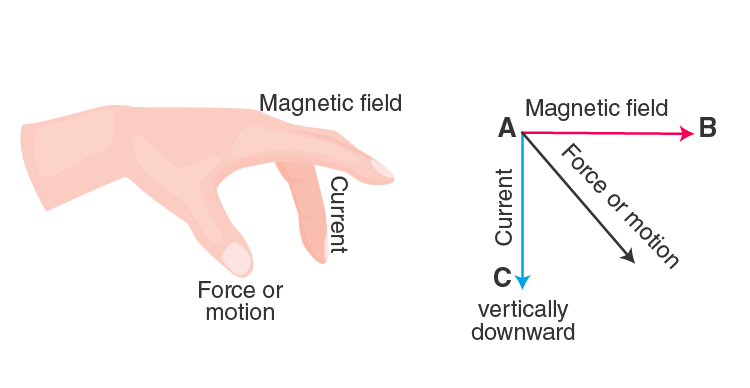Q19. What is the principle of an electric motor? Name some of the devices in which electric motors are used.

The principle of electric motor is based on the Fleming’s left hand rule. According to the law, hold the forefinger, the center finger, and the thumb of left hand at right angles to each other. The forefinger gives the direction of the magnetic field, the center finger gives the direction of current, and the thumb gives the direction of force acting on the conductor.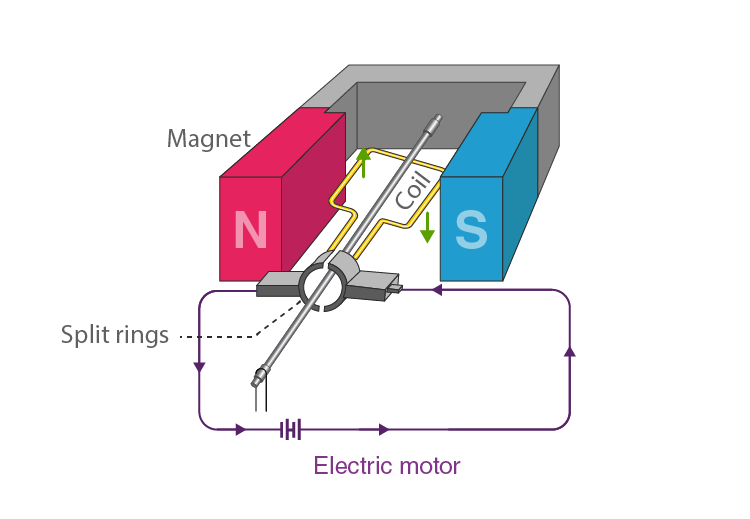Q20. a) In a DC motor, why must the current to the coil be reversed twice during each rotation?

b) What device reverses the current?

a) The current to the coil must be reversed twice during each rotation in a DC motor because by doing so the coil will keep rotating in the same direction.

b) Commutator.

Q21. a) State what would happen to the direction of rotation of a motor if:

i) the current were reversed

ii) the magnetic field were reversed

iii) both current and magnetic field were reversed simultaneously

b) In what ways can a motor be made more powerful?

a) i) If the current is reversed, then the direction of rotation would reverse

ii) If the magnetic field were reversed, then the direction of rotation would remain unchanged

iii) If both current and the magnetic field were reversed simultaneously then the direction of rotation would remain unchanged

b) A motor can be made powerful by winding the coil on to a soft iron core by increasing the number of turns of the coil.

Q22. a) What is an electric motor? With the help of a labelled diagram, describe the working of a simple electric motor.

b) What are the special features of commercial electric motors?

a) Electric motor is a device that is used for converting an electric energy into mechanical energy.

Working:The coil ABCD is placed in a horizontal position. The current enters the brush P which is a carbon and to the commutator which is a half ring X. The current produced in the coil ABCD leave the coil through the ring Y and brush Q. The direction of the magnetic field is from the N pole to the S pole. AB and CD move in the downward and the upward direction and this is based on the Fleming’s left hand rule which results in the anticlockwise movement of the coil.

When the brushes P and Q are in vertical position, they touch the gap between the two commutator rings and the current goes off. Because of the gained momentum, the coil continues to rotate when the current goes off. The side CD and AB comes to the left and right side respectively. As there is a change in the direction of the current, the change in the coil can also be seen.

b) In commercial electric motor:

i) to increase the strength of the magnetic field, the coil is wound on to a soft iron which makes the electric motor powerful

ii) insulated copper wire is used that has large number of turns

iii) a permanent magnet is replaced with an electromagnet

Multiple Choice Questions

Q23. In an electric motor, the direction of current in the coil changes once in each:

a) two rotations

b) one rotation

c) half rotation

d) one-fourth rotation

The correct option is c) half rotation

Q24. An electron beam enters a magnetic field at right angles to it as shown in the figure: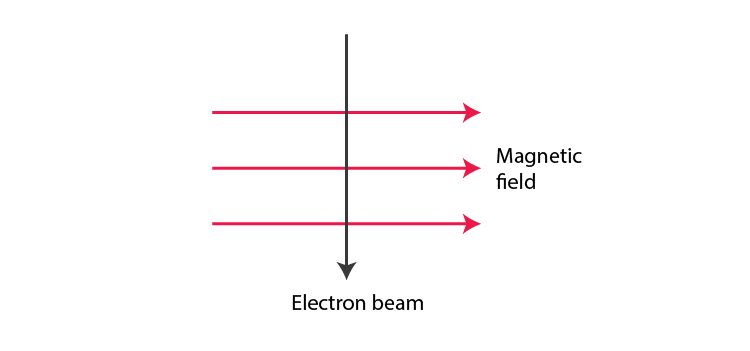The direction of force acting on the electron beam will be:

a) to the left

b) to the right

c) into the page

d) out of the page

The correct option is c) into the page

Q25. The force experienced by a current-carrying conductor placed in a magnetic field is the largest when the angle between the conductor and the magnetic field is:

a) 45

b) 60

c) 90

d) 180

The correct option is c) 90

Q26. The force exerted on a current-carrying wire placed in a magnetic field is zero when the angle between the wire and the direction of magnetic field is:

a) 45

b) 60

c) 90

d) 180

The correct option is c) 180

Q27. A current flows in a wire running between the S and N poles of a magnet lying horizontally shown  in the figure below: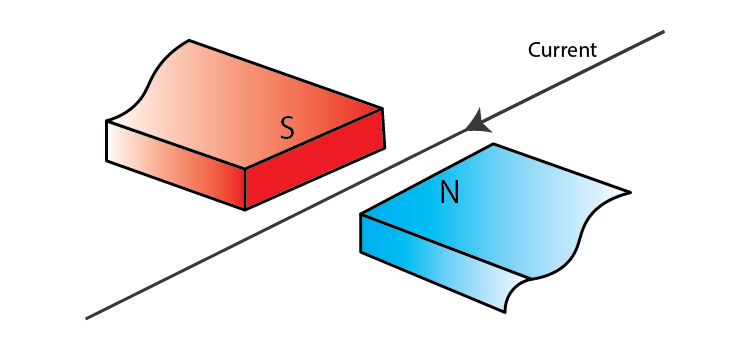The force on the wire due to the magnet is directed:

a) from N to S

b) from S to N

c) vertically downwards

d) vertically upwards

The correct option is c) vertically downwards

Q28. An electric motor is a device which transforms:

a) mechanical energy to electrical energy

b) heat energy to electrical energy

c) electrical energy to heat energy only

d) electrical energy to mechanical energy

The correct option is d) electrical energy to mechanical energy

Q29. A magnetic field exerts no force on:

a) an electric charge moving perpendicular to its direction

b) an unmagnetized iron bar

c) a stationary electric charge

d) a magnet

The correct option is c) a stationary electric charge

Q30. A horizontal wire carries a current as shown in figure below between magnetic poles N and S: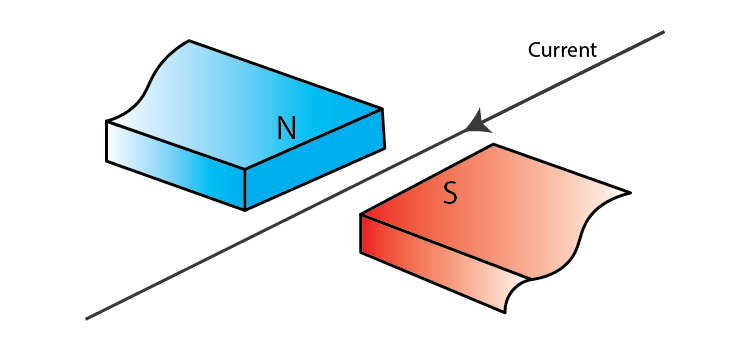Is the direction of the force on the wire due to the magnet:

a) in the direction of the current

b) vertically downwards

c) opposite to the current direction

d) vertically upwards

The correct answer is d) vertically upwards

Page No: 102

Q1. Name the device which converts mechanical energy into electric energy.

Electric generator is the device that is used for converting mechanical energy into electric energy.

Q2. Out of an AC generator and a DC generator:

a) which one uses a commutator (split rings)?

b) which one uses slip rings?

a) DC generator uses a commutator

b) AC generator uses slip rings

Q3. Name the phenomenon which is made use of in an electric generator,

Electromagnetic induction is the phenomenon which is made use of in an electric generator.

Q4. Name the rule which gives the direction of induced current.

Fleming’s right hand rule is used to get the direction of induced current.

Q5. What condition is necessary for the production of current by electromagnetic induction?

The relative motion between the wire and the magnet is the necessary condition for the production of current by an electromagnetic induction.

Q6. What type of generator is used at power stations?

AC generator is used at power stations.

Q7. What change should be made in an AC generator so that it may become a DC generator?

For an AC generator to become a DC generator, the slip rings must be replaced with a commutator.

Q8. State whether the following statements are true or false:

a) A generator works on the principle of electromagnetic induction.

b) A motor works on the principle of electromagnetic induction.

a) True

b) False

Q9. What is the function of brushes in an electric generator?

The function of the brushes in an electric generator is to transfer the current from the coil to the load.

Q10. When a wire is moved up and down in a magnetic field, a current is induced in the wire. What is this phenomenon known as?

The phenomenon in which the wire moves up and down in a magnetic field to induce the current in the wire is known as electromagnetic induction.

Q11. When current is ‘switched on’ and ‘switched off’ in a coil, a current is induced in another coil kept near it. What is this phenomenon known as?

The phenomenon in which current is switched on and off in a coil to induce current in another coil is known as electromagnetic induction.

Q12. What is the major difference between the simple alternator and most practical alternators?

Following is the major difference between the simple alternator and the most practical alternator:

Simple alternator has a magnet that is fixed with rotating coils

Practical alternator has a fixed coil with rotating magnets.

Q13. Why are thermal power stations usually located near a river?

The thermal power stations are usually located near a river because water is used in the making of the steam which is used for turning of the turbines.

Q14. List three sources of magnetic fields.

The three sources of magnetic fields are:

a) Wire carrying current

b) Electromagnet

c) Permanent magnet

Q15. Complete the following sentence:

A generator with commutator produces …… current.

Direct

Q16. Two circular coils A and B are placed closed to each other, if the current in the coil A is changed, will some current be induced in the coil B? Give reason for your answer.

Yes, when two coils are placed close to each other, the other coil will experience some current induced in it because of change in the magnetic field through the coil B. This is known as electromagnetic induction.

Q17. a) Explain the principle of an electric generator.

b) State two ways in which the current induced in the coil of a generator could be increased.

a) The principle of an electric generator is based on a straight conductor which is moved in a magnetic field such that current is induced in the conductor.

b) Following are the ways in which the current is induced in the coil of a generator:

i) By rotating the coil faster

ii) By using a coil with a larger area

Q18. a) What is the difference between alternating current and direct current?

b) What type of current is given by i) a dry cell ii) a power house generator?

a) i) The difference between alternating current and direct current is that alternating current can be reversed in direction at regular intervals of time while direct current flows in one direction.

ii) The value and direction in the DC current remains unchanged with the time while AC current changes with time and direction.

b) i) DC

ii) AC

Q19. State and explain Fleming’s right hand rule.

When a straight conductor is moving in a magnetic field, the direction of induced current is given by Fleming’s right hand rule.

The thumb, the fore finger, and the center finger of the right hand must be held at the right angles to one another. The forefinger shows the direction of the magnetic field, the thumb shows the direction of motion of conductor, and the center finger shows the direction of the induced current in the conductor.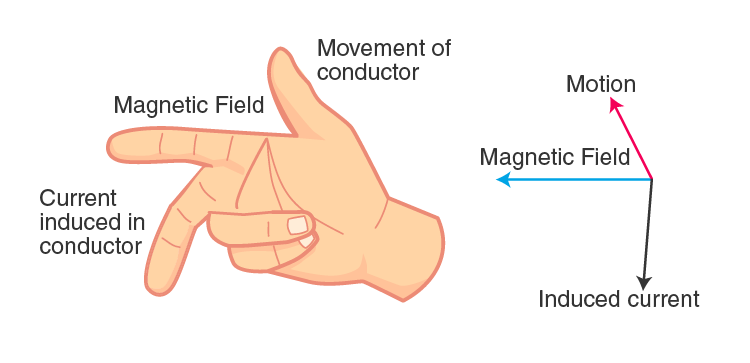Q20. Name and state the rule to find the direction of:

a) current induced in a coil due to its rotation in a magnetic field

b) force experienced by a current-carrying straight conductor placed in a magnetic field which is perpendicular to it.

a) Fleming’s right hand rule: The thumb, the forefinger, and the center finger of right hand are held perpendicular to each other. The thumb gives the direction of the motion of conductor, the forefinger gives the direction of magnetic field, and the center finger gives the direction of the induced current in the conductor.b) Fleming’s left hand rule: The forefinger, center finger, and the thumb of left hand are held at right angle to each other. The thumb gives the direction of force acting on the conductor, the forefinger gives the direction of the magnetic field and the center finger gives the direction of the current.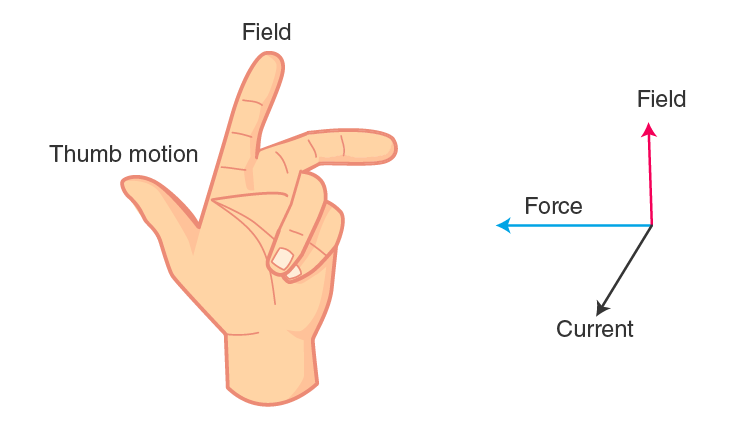Q21. a) In what respect does the construction of an AC generator differ from that of a DC generator?

b) What normally drives the alternators in a thermal power station? What fuels can be used to heat water in the boiler?

a) When it comes to the construction of the DC and AC generator, the ends of both the generator coils are linked to the outer circuit. In DC generator, the ends are connected to the commutator that has two half rings of copper. In AC generator, the ends of the coil are connected to the full rings of copper known as slip rings.

b) Thermal power stations use power of high pressure steam for heating water in the boiler and fuels such as coal

or natural gas is used.

Q22. Draw the labelled diagram of an AC generator. With the help of this diagram, explain the construction and working of an AC generator.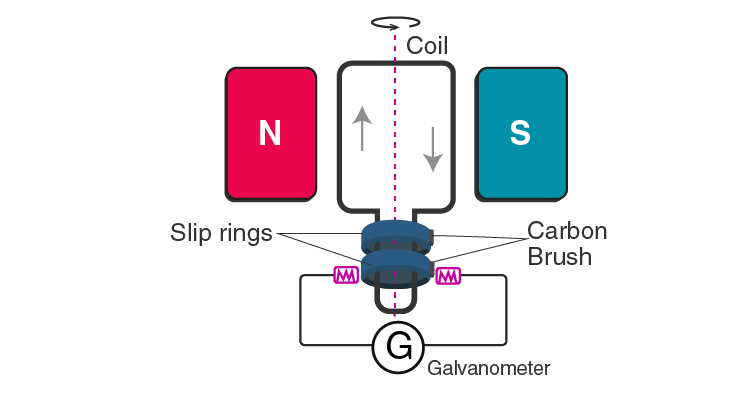Construction:

A simple AC generator consists of a rectangular coil ABCD which is rotated rapidly between the north and south poles of a strong horseshoe magnet. The magnet used is a permanent magnet M. Insulated copper wires are used for the making of the coil with large number of turns. The two ends A and D are connected to the metal slip rings R1 and R2. The contact between the slip rings is done with the help of the brushes B1 and B2. The brushes are connected to the galvanometer to show the flow of current in the external circuit.

Working:

When the coil ABCD is rotated in the anticlockwise direction, the AB side moves downward while the CD side moves upwards. Due to this movement there is induced current flow in the direction of BADC. In the first cycle, there is flow of current from the brush B1 to B2. In the second half cycle the position of AB and CD changes and the flow of current is from B2 to B1.

Q23. a) What do you understand by the term ‘electromagnetic induction’? Explain with the help of a diagram.

b) Name one device which works on the phenomenon of electromagnetic induction.

c) Describe different ways to induce current in a coil of wire.

a) Electromagnetic induction is the production of electricity from the magnetism.

The wire AB should be moved upwards between the poles of the horseshoe magnet. There is a deflection in the galvanometer when the wire is moved. This deflection shows that the current is produced in the wire AB. Therefore, when the wire is moved through the magnetic field, there is a production of electric current.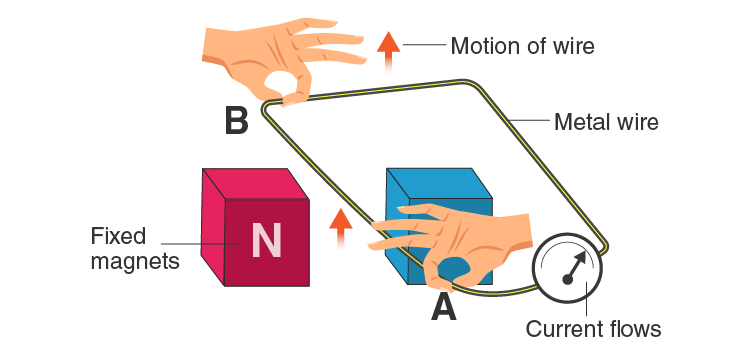b) Electric generator

c) Following are the different ways to induce current in the wire:

i) By moving the coil relative to the fixed magnet

ii) By moving the magnet relative to the fixed coil

Q24. a) What do you understand by the terms ‘direct current’ and ‘alternating current’?

b) Name some sources of direct current and some of alternating current.

c) State an important advantage of alternating current over direct current.

d) What is the frequency of AC supply in India?

a) When the current flows in just one direction, it is known as direct current while the flow of current in revsere direction at equal intervals of time is known as alternating current.

b) Sources of DC are: dry cell and car battery

Sources of AC are: AC generator and bicycle dynamos

c) An important advantage of AC over DC is that the transmission of AC over a long distances is much easier without much loss in electrical energy.

d) 50Hz.

Multiple Choice Questions

Q25. A rectangular coil of copper wire is rotated in a magnetic field. The direction of induced current changes once in each:

a) two revolutions

b) one revolution

c) half revolution

d) one-fourth revolution

The correct option is c) half revolution

Q26. The phenomenon of electromagnetic induction is:

a) the process of charging a body

b) the process of generating magnetic field due to a current passing through a coil

c) producing induced current in a coil due to relative motion between a magnet and the coil

d) the process of rotating a coil of an electric motor

The correct option is c) producing induced current in a coil due to relative motion between a magnet and the coil

Q27. The device used for producing electric current is called a:

a) generator

b) galvanometer

c) ammeter

d) motor

The correct option is a) generator

Q28. The essential difference between an AC generator and a DC generator is that:

a) AC generator has an electromagnet while a DC generator has permanent magnet

b) DC generator will generate a higher voltage

c) AC generator will generate a higher voltage

d) AC generator has slip rings while the DC generator has a commutator

The correct option is d) AC generator has slip rings while the DC generator has a commutator

Q29. When the switch S is closed in the figure given below, the pointer of the galvanometer moves to the right.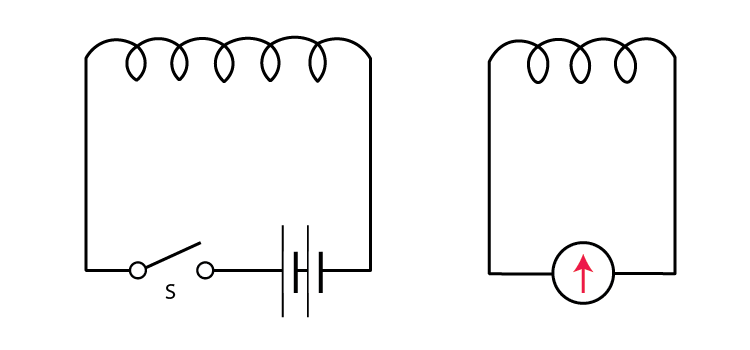If S is kept closed, will the pointer:

b) stay over on the right?

c) move to the left and stay there

d) move to and fro until S is opened

Q30. Each one of the following changes will increase emf in a simple generator except:

a) increasing the number of turns in the armature coil

b) winding the coil on a soft iron armature

c) increasing the size of the gap in which the armature turns

d) increasing the speed of rotation

The correct option is c) increasing the size of the gap in which the armature turns

Q31. The north pole of a long bar magnet was pushed slowly into a short solenoid connected to a galvanometer. The magnet was held stationary for a few seconds with the north pole in the middle of the solenoid and then withdrawn rapidly. The maximum deflection of the galvanometer was observed when the magnet was:

a) moving towards the solenoid

b) moving into solenoid

c)  at rest inside the solenoid

d) moving out of the solenoid

The correct option is d) moving out of the solenoid

Q32. An electric generator converts:

a) electrical energy into mechanical energy

b) mechanical energy into heat energy

c) electrical energy into chemical energy

d) heating effect of current

The correct option is d) heating effect of current

Q33. A d.c generator is based on the principle of:

a) electrochemical induction

b) electromagnetic induction

c) magnetic effect of current

d) heating effect of current

The correct option is b) electromagnetic induction

Q34. An induced current is produced when a magnet is moved into a coil. The magnitude of induced current does not depend on:

a) the speed with which the magnet is moved

b) the number of turns of the coil

c) the resistivity of the wire of the coil

d) the strength of the magnet

The correct option is c) the resistivity of the wire of the coil

Q35. The frequency of direct current is:

a) 0 Hz

b) 50 Hz

c) 60 Hz

d) 100 Hz

The correct option is a) 0 Hz

Q36. The frequency of alternating current supply in India is:

a) 0 Hz

b) 50 Hz

c) 60 Hz

d) 100 Hz

The correct option is b) 50 Hz

Page No: 113

Q1. What name is given to the device which automatically cuts off the electricity supply during short-circuiting in household wiring?

The device that is used to automatically cut off the electricity supply during the short-circuiting in the household wiring is known as electric fuse.

Q2. What is the usual capacity of an electric fuse used in the a) lighting circuit and b) in the power circuit of a small house?

Following is the usual capacity of an electric fuse that is used in:

a) 5A

b) 15A

Q3. Give the symbol of an electric fuse used in circuit diagram.

Following is the symbol of an electric fuse that is used in the circuit diagram: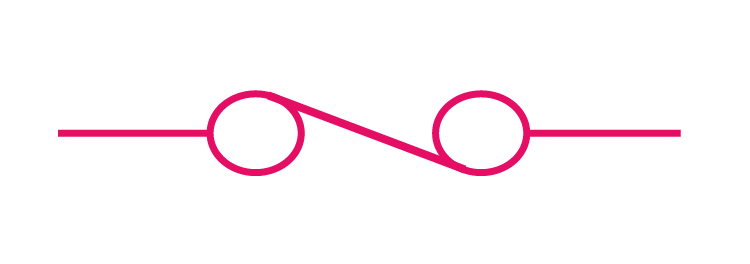Q4. State whether the following statements are true or false:

a) A wire with a green insulation is usually the live wire

b) A miniature circuit breaker (MCB) works on the heating effect of curreny

Following is the correct answers for:

a) False

b) False

Q5. Along with live wire and neutral wire, a third wire is also used in domestic electric wiring, what name is given to this third wire?

The third wire that is used along with live wire and neutral wire is the earth wire.

Q6. List the colours of the three wires in the cable connected to the plug of an electric iron.

Following are the colours of the three wires in the cable connected to the plug of an electric iron:

a) Red wire: live wire

b) Black wire: neutral wire

c) Green wire: Earth wire

Q7. What is the electric potential of the neutral wire in a mains supply cable?

The electric potential of the neutral wire in a mains supply cable is zero volt.

Q8. If fuses of 250mA, 500mA, 1A, 5A, and 10A were available, which one would be the most suitable for protecting an amplifier rated at 240V, 180W?

Given,

P = 180W

V = 240V

We know that,

P = VI

I = P/V = 180/240 = 0.75A

Therefore, the fuse wire should have the capacity to withstand a current of 0.75A. Therefore, 1A fuse is more suitable.

Q9. When does an electric short circuit occur?

For a short circuit to occur, the live wire and the neutral wire should come in contact with each other.

Q10. In which wire in an AC housing circuit is the switch introduced to operate the lights?

Live wire

Q11. In household circuit, is a fuse wire connected in series or in parallel?

In household circuit, a fuse wire is connected in series.

Q12. Usually three insulated wires of different colours are used in an electrical appliances. Name the three colours.

Following are the three colours that are used in an electric appliances:

a) Red

b) Black

c) Green

Q13. What is the usual colour of the insulation of a) live wire b) neutral wire c) earth wire?

a) Live wire: red

b) Neutral wire: black

c) Earth wire: green

Q14. What is the main purpose of earthing an electrical appliance?

The main purpose of earthing an electrical appliance is to avoid the risk of electric shocks.

Q15. Give two reasons why different electrical appliances in a domestic circuit are connected in parallel.

Following are the two reasons why different electrical appliances in a domestic circuit are connected in parallel:

a) When the appliances are connected in parallel, when one of them is switched off, the others keeping working.

b) All the appliances are working in the same voltage that is the mains supply voltage.

Q16. How should the electric lamps in a building be connected so that the switching on or off in a room has no effect on other lamps in the same building?

The lamps in a building should be connected in the parallel connection.

Q17. Fill in the following blanks with suitable words:

a) A fuse should always be placed in the …….. wire of a mains circuit.

b) The earth wire should be connected to the ….. of an appliance.

a) Live

b) Body.

Q18. a) Of what substance is the fuse wire made? Why?

b) Explain why a copper wire cannot be used as a fuse wire?

a) The substance that is used in the making of a fuse wire is tin-plated copper as it has a low melting point.

b) Copper wire cannot be used as a fuse wire because the melting point of the pure copper is high and this makes it difficult to melt during short circuits.

Q19. What type of electric fuse is used in electrical appliances like car stereos? Explain with the help of a labelled diagram.

Cartridge fuse is used in electrical appliances like car stereos. It consist of a glass tube T which is thin fuse wire that is sealed inside it. The glass tube has two metal caps at the ends. These two ends of the fuse are connected to the metal cap. These metals caps can be used for connecting the fuse in the circuit.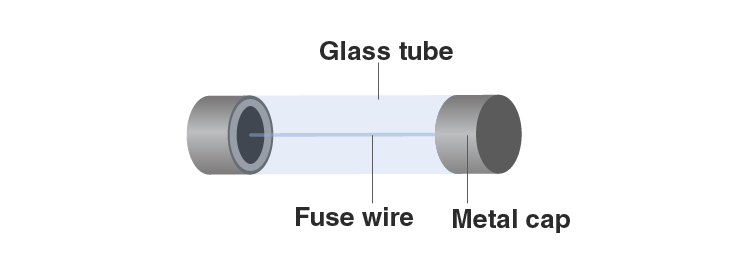Q20. Distinguish between the terms ‘overloading’ and ‘short-circuiting’ as used in domestic circuits.

When the live wire comes in contact with the neutral wire, it is known as short circuit.

When more than two electrical appliances with high power rating are switched on at the same time, the amount of current drawn by these appliances are large, this is known as overloading.

Q21. a) When does a fuse cut off current? How does it do it?

b) What is the maximum number of 60W bulbs that can be run from the mains supply of 220 volts if you do not want to overload a 5A fuse?

a) A fuse cut off current means when the current exceeds the safe vales and the fuse wire starts to melt and break the circuit. This is known as a fuse cut off current.

b) Given,

V = 220V

I = 5A

Let the maximum number of bulbs be y

Power of y bulbs, P = 60y

We know that,

P =VI

60y = 1100

y = 18.33

Therefore, the number of bulbs required are 18.

Q22. Explain the importance of using in a household electric circuit a) fuse b) earthing wire

a) A fuse is considered to be one of the important devices that is used for the protection so as to avoid the damages that happen due to either over load or short circuit.

b) An earthing wire is used as a electric shock resistant when a live wire comes in contact with the metal case of an electric appliance.

Q23. a) An electric iron is rated at 230V, 750W. Calculate i) the maximum current, ii) the number of units of electricity it would use in 30 minutes.

b) Which of the following fuse ratings would be suitable for this electric iron?

1A, 3A, 5A, 13A.

a) Given,

V = 230V

P = 750W

T = 30/60 = 0.5 hours

i) Let the maximum current be I

We know that,

P = VI

750 = 230I

I = 3.26A

ii) Electric energy consumed, E = PT

E = 0.75kW×0.5 hours

E = 0.375 kWh

Therefore, the number of units used in 30 minutes = 0.375

b) The maximum current for the given iron is 3.26A, therefore, 5A fuse rating will be suitable for the electric iron.

Q24. What is the function of an earth wire? Why is it necessary to earth the metallic bodies of electrical appliances?

The metallic bodies of an electrical appliances are earth to avoid the fatal electric shocks. When the live wire comes in direct contact with the metallic case, the amount of current that passing through the user body would be large. To avoid this, it is necessary that the electric appliances are earth.

Q25. a) What current is taken by a 3kW electric geyser working on 240V mains?

b) What size fuse should be used in the geyser circuit?

a) Given,

P = 3kW = 3000W

V = 240V

P = VI

I = P/V

I = 3000/240

I = 12.5A

b) The size of the fuse that should be used in the geyser circuit is 13A.

Q26. a) Why are fuses fitted in the fuse box of a domestic electricity supply?

b) What device could be used in place of the fuses?

a) The fuses fitted in the fuse box of a domestic electricity supply because to protect the wiring of the house from the excessive current flow in the circuit.

b) MCB

Q27. a) Draw a labelled diagram to show the domestic electric wiring from an electric pole to a room. Given the wiring for a bulb and a three-pin socket only.

b) State two hazards associated with the use of electricity.

c) State the important precautions which should be observed in the use of electricity.

d) What will you do if you see a person coming in contact with a live wire?

e) Explain why electric switches should not be operated with wet hands.

a)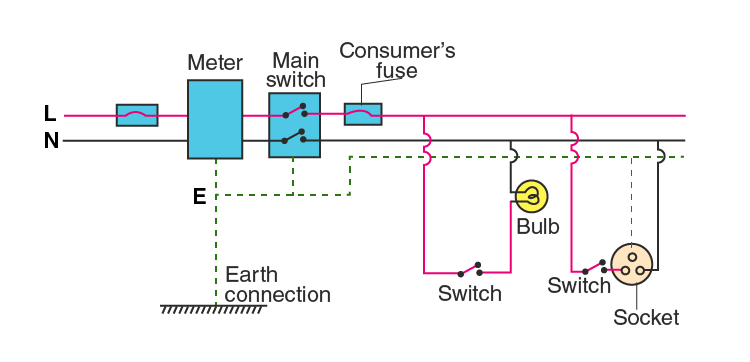b) Following are the two hazards that are associated with the use of electricity:

i) There are high chances of getting electric shock when a person touches a live electric wire.

ii) Due to faulty wire in the circuit, there is chance for short circuits.

c) Following are the important precautions that should be observed:

i) Using a good quality wires

ii) Proper earthing

iii) Usage of the electric appliances in dry condition only.

d) If a person comes in contact with the live wire then the mains must be switched off immediately so as to cut the supply of electricity.

e) Electric switches should not be operated when the hands are wet because we know that the water is a good conductor of electricity and we may get electric shock.

Multiple Choice Questions

Q28. At the time of short circuit, the current in the circuit:

a) reduces substantially

b) does not change

c) increases heavily

d) varies continuously

The correct option is c) increases heavily

Q29. A 1.25kW heater works on a 220V mains supply. What current rating would a suitable fuse have?

a) 2A

b) 5A

c) 10A

d) 13A

The correct option is c) 10A

Q30. The maximum number of 40W tube-lights connected in parallel which can safely be run from a 240V supply with a 5A fuse is:

a) 5

b) 15

c) 20

d) 30

The correct option is d) 30

Q31. In normal use, a current of 3.5A flows through a hair dryer. Choose a suitable fuse from the following:

a) 3A

b) 5A

c) 10A

d) 30A

The correct option is b) 5A

Q32. Which one of the following statements is not true?

a) In a house circuit, lamps are used in parallel

b) Switches, fuses, and circuit breakers should be placed in the neutral wire

c) An electric iron has its earth wire connected to the metal case to prevent the user receiving a shock

d) When connecting a three-core cable to a 13A three-pin plug, the red wire goes to the live pin

The correct option is b) switches, fuses, and circuit breakers should be placed in the neutral wire

Q33. A car headlamp of 48 W works on the car battery of 12V. The correct fuse for the circuit of this car headlamp will be:

a) 5A

b) 10A

c) 3A

d) 13A

The correct option is a) 5A

Q34. A 3-pin mains plug is fitted to the cable for a 1kW electric kettle to be used on a 250V a.c supply. Which of the following statement is not correct?

a) the fuse should be fitted in the live wire

b) a 13A fuse is the most appropriate value to use

c) the neutral wire is coloured black

d) the green wire should be connected to the earth pin

The correct option is b) a 13A fuse is the most appropriate value to use

Q35. A TV set consumes an electric power of 230 watts and runs on 230 volts mains aupply. The correct fuse for this TV set is:

a) 5A

b) 3A

c) 1A

d) 2A

The correct option is d) 2A

Q36. Circuit breaker device which can be used in place of fuse in domestic electric wiring is called:

a) CBD

b) DCB

c) MCD

d) MCB

The correct option is d) MCB

Q37. An MCB which cuts off the electricity supply in case of short-circuiting or overloading works on the:

a) chemical effect of current

b) heating effect of current

c) magnetic effect of current

d) electroplating effect of current